Key Publications
All Other Publications

Books and Surveys
 Computational Topology for Data Analysis. Tamal K. Dey, Yusu Wang. To be Published by Cambridge U. Press (2021 December). Details and Free Electronic Copy Order link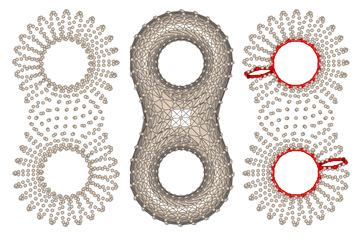Delaunay Mesh Generation. Siu-Wing Cheng, Tamal K. Dey, Jonathan R. Shewchuk. Published by CRC Press (2012 December). Details and Sample Chapters including a new algorithm for meshing 3D polyhedra with arbitrary small angles (chapter 9). Order link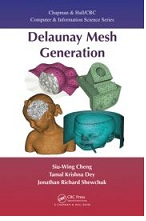Curve and Surface Reconstruction : Algorithms with Mathematical Analysis. T. K. Dey. Published by Cambridge University Press (2007). Preface and contents.  Two chapters. Erratum Order link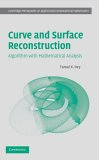Delaunay mesh generation of three dimensional domains. T. K. Dey. Technical Report OSU-CISRC-9/07-TR64, October, 2007. To appear in Tessellations in the sciences: Virtues, Techniques and Applications of Geometric Tilings, eds. R. van de Weygaert, G. Vegter, J. Ritzerveld & V. Icke, Springer-Verlag, 2009. Delaunay meshes are used in various applications such as finite element analysis, computer graphics rendering, geometric modeling, and shape analysis. As the applications vary, so do the domains to be meshed. Although meshing of geometric domains with Delaunay simplices have been around for a while, provable techniques to meshing various types of three dimenisonal domains have been developed only recently. We devote this article to presenting these techniques. We survey various related results and detail a few core algorithms that have provable guarantees and are amenable to practical implementation. Delaunay refinement, a paradigm originally developed for guaranteeing shape quality of mesh elements, is a common thread in these algorithms. We finish the article by listing a set of open questions.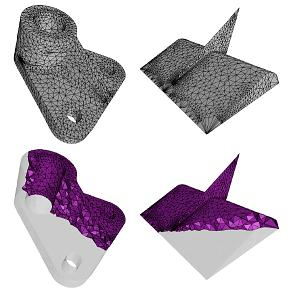Delaunay Mesh Genration of Surfaces T. K. Dey. Oberwolfach Report on Triangulations, Report No. 24/2012, Page 1447.
 Sample based geometric modeling T. K. Dey. Chapter in Geometric and Algorithmic Aspects of Computer-Aided Design and Manufacturing, eds Janardan, Smid, Dutta. DIMACS series in Discrete Mathematics and Theoretical Computer Science, Volume 67, 2005. A new paradigm for digital modeling of physical objects from sample points is fast emerging due to recent advances in scanning technology. Researchers are investigating many of the traditional modeling problems in this new setting. We name this new area as sample based geometric modeling. The purpose of this article is to expose the readers to this new modeling paradigm through three basic problems, namely surface reconstruction, medial axis approximation, and shape segmentation. The algorithms for these three problems developed by the author and his co-authors are described.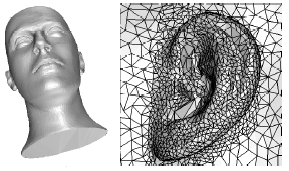Curve and surface reconstruction T. K. Dey. Chapter in Handbook of Discrete and Computational Geometry, Goodman and O' Rourke eds., CRC press, 2nd edition (2004) New version for 3rd edition to be published (2016). The problem of reconstructing a shape from its sample appears in many scientific and engineering applications. Because of the variety in shapes and applications, many algorithms have been proposed over the last two decades, some of which exploit application-specific information and some of which are more general. We will concentrate on techniques that apply to the general setting and have proved to provide some guarantees on the quality of reconstruction.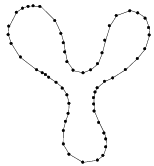Papers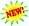Computing optimal persistent cycles for levelset zigzag on manifold-like complexes T. K. Dey, T. Hou,  arXiv: https://arxiv.org/abs/2105.00518 May 2021. In standard persistent homology, a persistent cycle born and dying with a persistence interval (bar) associates the bar with a concrete topological representative, which provides means to effectively navigate back from the barcode to the topological space. Among the possibly many, optimal persistent cycles bring forth further information due to having guaranteed quality. However, topological features usually go through variations in the lifecycle of a bar which a single persistent cycle may not capture. Hence, for persistent homology induced from PL functions, we propose levelset persistent cycles consisting of a sequence of cycles that depict the evolution of homological features from birth to death. Our definition is based on levelset zigzag persistence which involves four types of persistence intervals as opposed to the two types in standard persistence. For each of the four types, we present a polynomial-time algorithm computing an optimal sequence of levelset persistent pp-cycles for the so-called weak (p+1)(p+1)-pseudomanifolds. Given that optimal cycle problems for homology are NP-hard in general, our results are useful in practice because weak pseudomanifolds do appear in applications. Our algorithms draw upon an idea of relating optimal cycles to min-cuts in a graph that we exploited earlier for standard persistent cycles. Note that levelset zigzag poses non-trivial challenges for the approach because a sequence of optimal cycles instead of a single one needs to be computed in this case.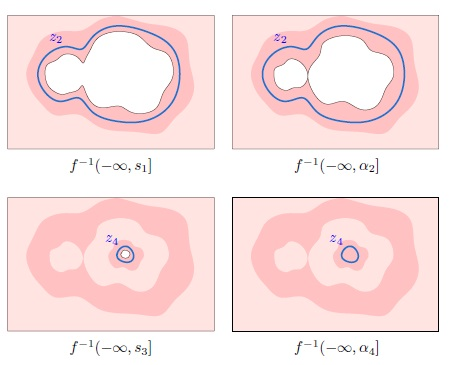Computing zigzag persistence on graphs in near-linear time T. K. Dey, T. Hou,  March 2021, Proc. Internat. Sympos. Comput. Geom. (2021) (SoCG 2021). Graphs model real-world circumstances in many applications where they may constantly change to capture the dynamic behavior of the phenomena. Topological persistence which provides a set of birth and death pairs for the topological features is one instrument for analyzing such changing graph data. However, standard persistent homology defined over a growing space cannot always capture such a dynamic process unless shrinking with deletions is also allowed. Hence, zigzag persistence which incorporates both insertions and deletions of simplices is more appropriate in such a setting. Unlike standard persistence which admits nearly linear-time algorithms for graphs, such results for the zigzag version improving the general $O(m^w)$ time complexity are not known, where $w< 2.37286$ is the matrix multiplication exponent. In this paper, we propose algorithms for zigzag persistence on graphs which run in near-linear time. Specifically, given a filtration with $m$ additions and deletions on a graph with $n$ vertices and edges, the algorithm for $0$-dimension runs in $O(m\log^2 n+m\log m)$ time and the algorithm for 1-dimension runs in $O(m\log^4 n)$ time.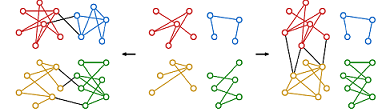Persistence of the Conley Index in Combinatorial Dynamical Systems T. K. Dey, M. Mrozek, and R. Slechta,  February 2020, Proc. Internat. Sympos. Comput. Geom. (2020) (SoCG 2020).A combinatorial framework for dynamical systems provides an avenue for connecting classical  dynamics with algorithmic methods. Discrete Morse vector fields by Forman and its recent adaptation to multivector fields by Mrozek have laid the foundation for this combinatorial framework. In this work, we make a further connection to computational topology by putting the well known Conley index of (multi)vector fields into the persistence framework. Conley indices are homological features of the so called invariant sets in a dynamical system. We show how one can compute the persistence of these indices over a sequence of multivector fields sampled from an underlying dynamical system. This also enables us to track' features in a dynamical system in a principled way.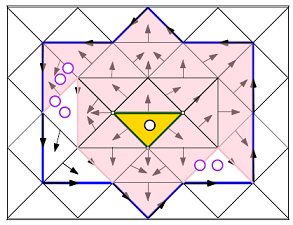An efficient algorithm for 1-dimensional (persistent) path homology T. K. Dey, T. Li, and Y. Wang, January 2020, arxiv: Proc. Internat. Sympos. Comput. Geom. (2020) (SoCG 2020).This paper focuses on developing an efficient algorithm for analyzing a directed network (graph) from a topological viewpoint. A prevalent technique for such topological analysis involves computation of homology groups and their persistence. These concepts are well suited for spaces that are not directed. As a result, one needs a concept of homology that accommodates orientations in input space. Path-homology developed for directed graphs by Grigor'yan, Lin, Muranov and Yau has been effectively adapted for this purpose recently by Chowdhury and M�moli. They also give an algorithm to compute this path-homology. Our main contribution in this paper is an algorithm that computes this path-homology and its persistence more efficiently for the 11-dimensional (H_1H1) case. In developing such an algorithm, we discover various structures and their efficient computations that aid computing the 1-dimensional path-homnology. We implement our algorithm and present some preliminary experimental results.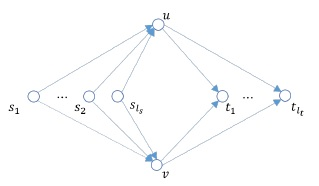Road Network reconstruction from Satellite Images with Machine Learning Supported by Topological Methods T. K. Dey, J. Wang, and Y. Wang, September 2019, arxiv:https://arxiv.org/pdf/1909.06728.pdf   A shoter version to appear in SIGSPATIAL 2019.Automatic Extraction of road network from satellite images is a goal that can benefit and even enable new technologies. Methods that combine machine learning (ML) and computer vision have been proposed in recent years which make the task semi-automatic by requiring the user to provide curated training samples. The process can be fully automatized if training samples can be produced algorithmically. Of course, this requires a robust algorithm that can reconstruct the road networks from satellite images reliably so that the output can be fed as training samples. In this work, we develop such a technique by infusing a persistence-guided discrete Morse based graph reconstruction algorithm into ML framework.We elucidate our contributions in two phases. First, in a semi-automatic framework, we combine a discrete-Morse based graph reconstruction algorithm with an existing CNN framework to segment input satellite images. We show that this leads to reconstructions with better connectivity and less noise. Next, in a fully automatic framework, we leverage the power of the discrete-Morse based graph reconstruction algorithm to train a CNN from a collection of images without labelled data and use the same algorithm to produce the final output from the segmented images created by the trained CNN. We apply the discrete-Morse based graph reconstruction algorithm iteratively to improve the accuracy of the CNN. We show promising experimental results of this new framework on datasets from SpaceNet Challenge.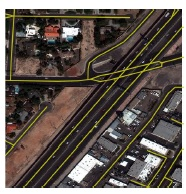T. K. Dey and Cheng Xin ., May 2019, arxiv: https://arxiv.org/abs/1904.03766The classical persistence algorithm virtually computes the unique decomposition of a persistence module implicitly given by an input simplicial filtration. Based on matrix reduction, this algorithm is a cornerstone of the emergent area of topological data analysis. Its input is a simplicial filtration defined over integers $\mathbb{Z}$ giving rise to a $1$-parameter persistence module. It has been recognized that multi-parameter version of persistence modules given by simplicial filtrations over $d$-dimensional integer grids $\mathbb{Z}^d$ is equally or perhaps more important in data science applications. However, in the multi-parameter setting, one of the main bottlenecks is that topological summaries such as barcodes and distances among them cannot be as efficiently computed as in the $1$-parameter case because there is no known generalization of the persistence algorithm for computing the decomposition of multi-parameter persistence modules. The Meataxe algorithm, a popular one known for computing such a decomposition runs in $\tilde{O}(n^{12d})$ time where $n$ is the size of the input filtration. We present for the first time a generalization of the persistence algorithm based on a generalized matrix reduction technique that runs in $O(n^{2\omega+1})$  time for $d=2$ and in $O(n^{d(2\omega + 1)})$ time for $d>2$ where $\omega<2.373$ is the exponent for matrix multiplication. Various structural and computational results connecting the graded modules from commutative algebra to matrix reductions are established through the course.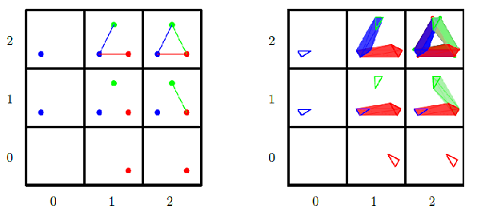Computing Minimal Persistent Cycles: Polynomial and Hard Cases T. K. Dey, T. Hou, and S. Mandal.   Proceedings ACM-SIAM Sympos. Discrete Algorithms (SODA 20), to appear.July 2019, Persistent cycles, especially the minimal ones, are useful geometric features functioning as augmentations for the intervals in the purely topological persistence diagrams (also termed as barcodes). In our earlier work, we showed that computing minimal 1-dimensional persistent cycles (persistent 1-cycles) for finite intervals is NP-hard while the same for infinite intervals is polynomially tractable. In this paper, we address this problem for general dimensions with Z2 coefficients. In addition to proving that it is NP-hard to compute minimal persistent d-cycles (d>1) for both types of intervals given arbitrary simplicial complexes, we identify two interesting cases which are polynomially tractable. These two cases assume the complex to be a certain generalization of manifolds which we term as weak pseudomanifolds. For finite intervals from the d-th persistence diagram of a weak (d+1)-pseudomanifold, we utilize the fact that persistent cycles of such intervals are null-homologous and reduce the problem to a minimal cut problem. Since the same problem for infinite intervals is NP-hard, we further assume the weak (d+1)-pseudomanifold to be embedded in  R^{d+1}Rd+1 so that the complex has a natural dual graph structure and the problem reduces to a minimal cut problem. Experiments with both algorithms on scientific data indicate that the minimal persistent cycles capture various significant features of the data.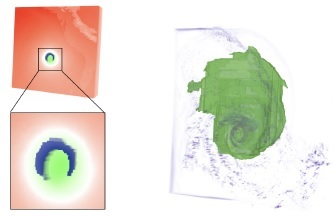Petsistent 1-Cycles: Definition, Computation, and Its Application T. K. Dey , T. Hou, and S. Mandal . Proceedings  Computational Topology in Image Context (CTIC 2019),  LNCS, Vol. 11382, pages 123--136.arxiv version: https://arxiv.org/abs/1810.04807See this web-page for software etc.: http://web.cse.ohio-state.edu/~dey.8/PersLoop/Persistence diagrams, which summarize the birth and death of homological features extracted from data, are employed as stable signatures for applications in image analysis and other areas. Besides simply considering the multiset of intervals included in a persistence diagram, some applications need to find representative cycles for the intervals. In this paper, we address the problem of computing these representative cycles, termed as persistent $1$-cycles. The definition of persistent cycles is based on the interval module decomposition of persistence modules, which reveals the structure of persistent homology. After showing that the computation of the optimal persistent $1$-cycles is NP-hard,  we propose an alternative set of  meaningful persistent $1$-cycles that can be computed with an efficient polynomial time algorithm. We also inspect the stability issues of the optimal persistent $1$-cycles and the persistent $1$-cycles computed by our algorithm with the observation that the perturbations of both cannot be properly bounded. We design a software which applies our algorithm to various datasets. Experiments on 3D point clouds, mineral structures, and images show the effectiveness of our algorithm in practice.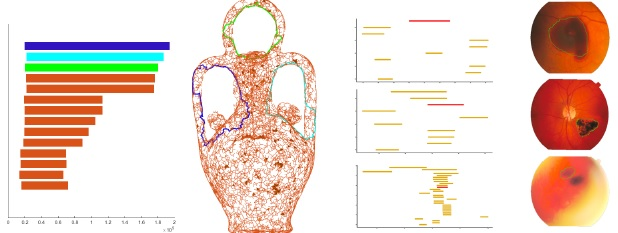T. K. Dey , R. Slechta.  Internat. Conf. Discrete Geoem. for Comput. Imagery (DGCI 2019), pages 89--100 .arxiv version: https://arxiv.org/abs/1810.04388See this web-page for software: https://github.com/rslechta/pers-contract/Persistent homology is a popular data analysis technique that is used to capture the changing topology of a filtration associated with some simplicial complex $K$. These topological changes are summarized in persistence diagrams. We propose two contraction operators which when applied to $K$ and its associated filtration, bound the perturbation in the persistence diagrams. The first assumes that the underlying space of $K$ is a $2$-manifold and ensures that simplices are paired with the same simplices in the contracted complex as they are in the original. The second is for arbitrary $d$-complexes, and bounds the bottleneck distance between the initial and contracted $p$-dimensional persistence diagrams. This is accomplished by defining interleaving maps between persistence modules which arise from chain maps defined over the filtrations. In addition, we show how the second operator can efficiently compose across multiple contractions. We conclude with experiments demonstrating the second operator's utility on manifolds.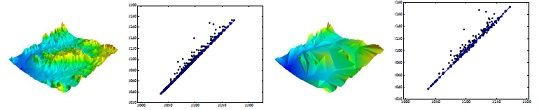Computing height persistence and homology generators in R^3 efficiently T. K. Dey , Proc. 30th ACM-SIAM Sympos. Discrete Algorithms, 2019 (SODA 19), pages 2649--2662.arxiv version: https://arxiv.org/abs/1807.03655Talk SlidesRecently it has been shown that computing the dimension of the   first homology group $H_1(K)$ of a simplicial $2$-complex $K$  embedded linearly in $R^4$ is as hard as computing the rank of a sparse $0-1$   matrix. This puts a major roadblock to computing persistence and a homology basis (generators) for complexes embedded in $R^4$  and beyond in less than quadratic or even near-quadratic time. But, what about dimension three? It is known that persistence for piecewise linear functions on a complex $K$ with $n$ simplices can be computed in $O(n\log n)$ time and a set of generators of total size $k$ can be computed in $O(n+k)$ time when $K$ is a graph or a surface linearly embedded in $R^3$. But, the question for general simplicial complexes $K$  linearly embedded in $R^3$ is not completely settled. No algorithm with a complexity better than that of  the matrix multiplication is known for this important case. We show that the persistence for {\em height functions} on such complexes, hence called {\em height persistence}, can be computed in $O(n\log n)$ time. This allows us to compute a basis (generators) of $H_i(K)$, $i=1,2$, in $O(n\log n+k)$ time where $k$ is the size of the output. This improves significantly the current best bound of $O(n^{\omega})$, $\omega$ being the matrix multiplication exponent. We achieve these improved bounds by leveraging recent results on zigzag persistence in computational topology, new observations about Reeb graphs, and some efficient geometric data structures.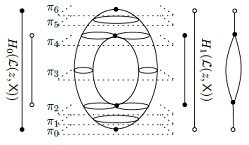T. K. Dey and R. Slechta. Proc. SMI 2018,  Computers & Graphics, .Vol. 74, 33-43.Journal version: https://doi.org/10.1016/j.cag.2018.05.002Recently, discrete Morse vector fields have been shown to be useful in various applications. Analogous to the simplification of large meshes using edge contractions, one may want to simplify the cell complex $K$ on which a discrete Morse vector field $V(K)$ is defined. To this end, we define a gradient aware edge contraction operator for triangulated $2$-manifolds with the following guarantee. If $V(K)$ was generated by a specific persistence-based method, then the vector field that results from our contraction operator is exactly the same as the vector field produced by applying the same persistence-based method to the contracted complex. An implication of this result is that local operations on $V(K)$ are sufficient to produce the persistence-based vector field on the contracted complex. Furthermore, our experiments show that the structure of the vector field is largely preserved by our operator. For example, $1$-unstable manifolds remain largely unaffected by the contraction. This suggests that for some applications of discrete Morse theory, it is sufficient to use a contracted complex.Also, see our recent work on Discrete Morse based reconstruction here and here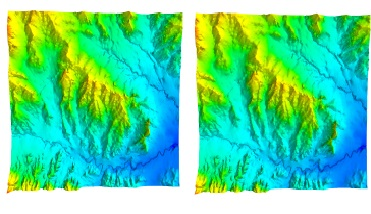T. K. Dey, M. Juda, T. Kapela, J. Kubica, M. Lipinski, M. Mrozek.  https://arxiv.org/abs/1801.06590. 2018.  SIAM J. on Applied Dynamical System,  Vol. 18, Issue 1, 510--530, 2019. We investigate combinatorial dynamical systems on simplicial complexes considered as finite topological spaces.  Such systems arise in a natural way from sampling dynamics and may be used to reconstruct some features  of the dynamics directly from the sample.  We study the homological persistence of Morse decompositions of such systems,  an important descriptor of the dynamics, as a tool for validating the reconstruction. Our framework can be viewed as a step toward extending the classical persistence theory to vector cloud" data.  We present experimental results on two numerical examples.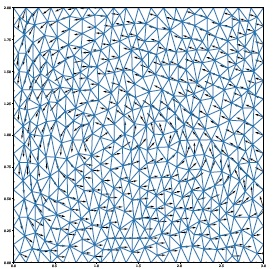Here is the n-D version of the SoCG paper.Computing Bottelneck Distance for Multi-parameter Interval Decomposable Persistence ModulesPreprint, September, 2019. Computing Bottleneck Distance for 2-D Interval Decomposable Modules T. K. Dey, C. Xin. Proc. 34th Internat. Sympos. Comput. Geoem., 32:1--32:15 (SoCG 2018).Computation of the interleaving distance between persistence modules is a central task in topological data analysis. For $1$-D persistence modules, thanks to the isometry theorem, this can be done by computing the bottleneck distance with known efficient algorithms. The question is open for most $n$-D persistence modules, $n>1$, because of the well recognized complications of the indecomposables. Here, we consider a reasonably complicated class called {\em $2$-D interval decomposable} modules whose indecomposables may have a description of non-constant complexity. We present a polynomial time algorithm to compute the bottleneck distance for these modules from indecomposables, which bounds the interleaving distance from above, and give another algorithm to compute a new distance called {\em dimension distance} that bounds it from below.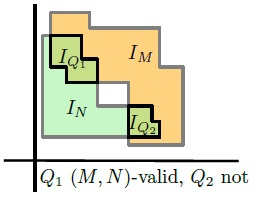Graph Reconstruction by Discrete Morse Theory T. K. Dey, J. Wang and Y. Wang.  Proc. Internat. Sympos. Comput. Geom., 31:1--31:15 (SoCG 2018).Recovering hidden graph-like structures from potentially noisy data is a fundamental task in modern data analysis. Recently, a persistence-guided discrete Morse-based framework to extract a geometric graph from low-dimensional data has become popular. However, to date, there is very limited theoretical understanding of this framework in terms of graph reconstruction. This paper makes a first step towards closing this gap. Specifically, first, leveraging existing theoretical understanding of persistence-guided discrete Morse cancellation, we provide a simplified version of the existing discrete Morse-based graph reconstruction algorithm. We then introduce a simple and natural noise model and show that the aforementioned framework can correctly reconstruct a graph under this noise model, in the sense that it has the same loop structure as the hidden ground-truth graph, and is also geometrically close. We also provide some experimental results for our simplified graph-reconstruction algorithm. Also see this paper in SIGSPATIAL (2017).Improved Road Network Reconstruction Using Discrete Morse Theory. T. K. Dey, J. Wang, and Y. Wang. Proc. SIGSPATIAL 2017.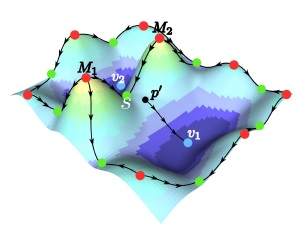Application of  Topological Data Analysis in Machine Learning for  Image and Protein Classification
 Protein Classification with Improved Topological Data AnalysisT. K. Dey and S. Mandal. Proc. Workshop on Algorithms in Bioinformatics (WABI 2018), 6:1--6:13. DOI 10.4230/LIPIcs.WABI.2018.6Web-page: http://web.cse.ohio-state.edu/~dey.8/proteinTDA/Automated annotation and analysis of protein molecules have long been a topic of interest due to immediate applications in medicine and drug design. In this work, we propose a topology based, fast, scalable, and parameter-free technique to generate protein signatures. We build an initial simplicial complex using information about the protein’s constituent atoms, including radius and existing chemical bonds, to model the hierarchical structure of the molecule. Simplicial collapse is used to construct a filtration which we use to compute persistent homology. This information constitutes our signature for the protein. In addition, we demonstrate that this technique scales well to large proteins. Our method shows sizable time and memory improvements compared to other topology based approaches. We use the signature to train a protein domain classifier. Finally, we compare this classifier against models built from state-of-the-art structure- based protein signatures on standard datasets to achieve a substantial improvement in accuracy. Improved Image Classification using Topological Persistence T. K. Dey, S. Mandal, W. Varcho.  Proc. Vision Modeling and Visualization.,  (VMV 2017). Image classification has been a topic of interest for many years. With the advent of Deep Learning, impressive progress has been made on the task, resulting in quite accurate classification. Our work focuses on improving modern image classification techniques by considering topological features as well. We show that incorporating this information allows our models to improve the accuracy, precision and recall on test data, thus providing evidence that topological signatures can be leveraged for enhancing some of the state-of-the art applications in computer vision.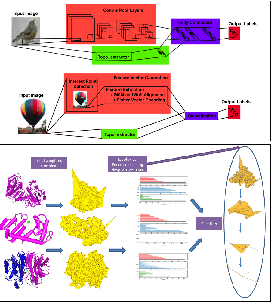T. K. Dey, T. Li, and Y. Wang. Proc. LATIN 2018: Theoretical Informatics, LNCS, Vol. 10807, 376--398 (LATIN 2018).Efficient computation of shortest cycles which form a homology basis under Z2-additions in a given simplicial complex K has been researched actively in recent years. When the complex K is a weighted graph with n vertices and m edges, the problem of computing a shortest (homology) cycle basis is known to be solvable in $O(m^2n/\log n+ n^2m)$-time. Several works [1,2] have addressed the case when the complex K is a 2-manifold. The complexity of these algorithms depends on the rank g of the one-dimensional homology group of K. This rank g has a lower bound of $\Theta(n)$, where n denotes the number of simplices in K giving an $O(n^4)$ worst-case time complexity for the algorithms in [1,2]. This worst-case complexity is improved in  to $O(n^\omega+n^2g^{\omega-1})$ for general simplicial complexes where $\omega< 2.3728639$  is the matrix multiplication exponent. Taking $g=\Theta(n)$, this provides an $O(n^{\omega+1})$ worst-case algorithm. In this paper, we improve this time complexity. Combining the divide and conquer technique from  with the use of annotations from , we present an algorithm that runs in $O(n^\omega+n^2g)$ time giving the first $O(n^3)$ worst-case algorithm for general complexes. If instead of minimal basis, we settle for approximate basis, we can improve the running time even further. We show that a 2-approximate minimal homology basis can be computed in $O(n^{\omega}\sqrt{n \log n})$ expected time. We also study more general measures for defining the minimal basis and identify reasonable conditions on these measures that allow computing a minimal basis efficiently.
 Temporal Hierarchical Clustering T. K. Dey, A. Rossi, and A. Sidiropoulos. Proc.  28th Internat. Symposium on Algorithms and Computation (ISAAC 2017). We study hierarchical clusterings of metric spaces that change over time. This is a natural geometric primitive for the analysis of dynamic data sets. Specifically, we introduce and study the problem of finding a temporally coherent sequence of hierarchical clusterings from a sequence of unlabeled point sets. We encode the clustering objective by embedding each point set into an ultrametric space, which naturally induces a hierarchical clustering of the set of points. We enforce temporal coherence among the embeddings by finding correspondences between successive pairs of ultrametric spaces which exhibit small distortion in the Gromov-Hausdorff sense. We present both upper and lower bounds on the approximability of the resulting optimization problems.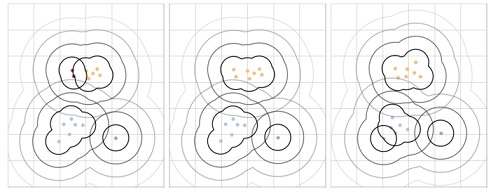Temporal Clustering T. K. Dey, A. Rossi, and A. Sidiropoulos. Proc. European Symposium on Algorithms (ESA 2017). Full Version https://arxiv.org/abs/1704.05964 We study the problem of clustering sequences of unlabeled point sets taken from a common metric space. Such scenarios arise naturally in applications where a system or process is observed in distinct time intervals, such as biological surveys and contagious disease surveillance. In this more general setting existing algorithms for classical (i.e.~static) clustering problems are not applicable anymore. We propose a set of optimization problems which we collectively refer to as \emph{temporal clustering}. The quality of a solution to a temporal clustering instance can be quantified using three parameters: the number of clusters $k$, the spatial clustering cost $r$, and the maximum cluster displacement $\delta$ between consecutive time steps. We consider spatial clustering costs which generalize the well-studied $k$-center, discrete $k$-median, and discrete $k$-means objectives of classical clustering problems. We develop new algorithms that achieve trade-offs between the three objectives $k$, $r$, and $\delta$. Our upper bounds are complemented by inapproximability results.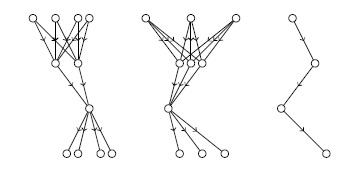Topological analysis of nerves, Reeb spaces, mappers, and multiscale mappers T. K. Dey, F. Memoli, and Y. Wang. Proc. Internat. Sympos. Comput. Geom. (2017) (SoCG 2017). Full Version [talk slides] Data analysis often concerns not only the space where data come from, but also various types of maps attached to data. In recent years, several related structures have been used to study maps on data, including Reeb spaces, mappers and multiscale mappers. The construction of these structures also relies on the so-called \emph{nerve} of a cover of the domain.      In this paper, we aim to analyze the topological information encoded in these structures in order to provide better understanding of these structures and facilitate their practical usage.      More specifically, we show that the one-dimensional homology of the nerve complex $N(\mathcal{U})$ of a path-connected cover $\mathcal{U}$ of a domain $X$ cannot be richer than that of the domain $X$ itself. Intuitively, this result means that no new $H_1$-homology class can be created'' under a natural map from $X$ to the nerve complex $N(\mathcal{U})$. Equipping $X$ with a pseudometric $d$, we further refine this result and characterize the classes of $H_1(X)$ that may survive in the nerve complex using the notion of \emph{size} of the covering elements in $\mathcal{U}$. These fundamental results about nerve complexes then lead to an analysis of the $H_1$-homology of Reeb spaces, mappers and multiscale mappers.      The analysis of $H_1$-homology groups unfortunately does not extend to higher dimensions. Nevertheless, by using a map-induced metric, establishing a Gromov-Hausdorff convergence result between mappers and the domain, and interleaving relevant modules, we can still analyze the persistent homology groups of (multiscale) mappers to establish a connection to Reeb spaces.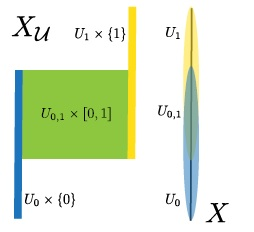Declutter and resample: Towards parameter free denoising M. Buchet, T. K. Dey, J. Wang, and Y. Wang.  Proc. Internat. Sympos. Comput. Geom. (2017), (SoCG 2017). Full Version [talk slides] In many data analysis applications the following scenario is commonplace: we are given a point set that is supposed to sample a hidden ground truth $K$ in a metric space, but it got corrupted with noise so that some of the  data points lie far away from $K$ creating outliers also termed as {\em ambient noise}. One of the main goals of denoising algorithms is to eliminate such noise so that the curated data lie within a bounded Hausdorff distance of $K$. Popular denoising approaches such as deconvolution and thresholding often require the user to set several parameters and/or to choose an appropriate noise model while guaranteeing only asymptotic convergence. Our goal is to lighten this burden as much as possible while ensuring theoretical guarantees in all cases. Specifically, first, we propose a simple denoising algorithm that requires only a single parameter but provides a theoretical guarantee on the quality of the output on general input points. We argue that this single parameter cannot be avoided. We next present a simple algorithm that avoids even this parameter by paying for it with a slight strengthening of the sampling condition on the input points which is not unrealistic. We also provide some preliminary empirical evidence that our algorithms are effective in practice.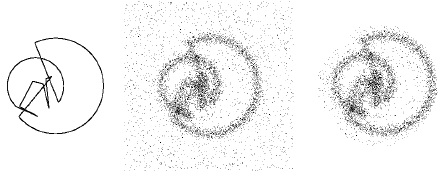Parameter-free topology inference and sparsification for data on manifolds T. K. Dey, Z. Dong, and Y. Wang. (2015), older version at arXiv:1505.06462. Proc. ACM-SIAM Sympos. Discrete Algorithms (SODA 2017). [talk slides] In topology inference from data, current approaches face two major problems. One concerns the selection of a correct parameter to build an appropriate complex on top of the data points; the other involves with the typical large' size of this complex. We address these two issues in the context of inferring homology from sample points of a smooth manifold of known dimension sitting in an Euclidean space Rk. We show that, for a sample size of n n points, we can identify a set of O(n2)O(n^2) points (as opposed to O(n⌈k2⌉)O(n^\ceil{k/2}) Voronoi vertices) approximating a subset of the medial axis that suffices to compute a distance sandwiched between the well known local feature size and the local weak feature size (in fact, the approximating set can be further reduced in size to O(n) O(n)). This distance, called the lean feature size, helps pruning the input set at least to the level of local feature size while making the data locally uniform. The local uniformity in turn helps in building a complex for homology inference on top of the sparsified data without requiring any user-supplied distance threshold. Unlike most topology inference results, ours does not require that the input is dense relative to a {\em global} feature such as {\em reach} or {\em weak feature size}; instead it can be adaptive with respect to the local feature size. We present some empirical evidence in support of our theoretical claims.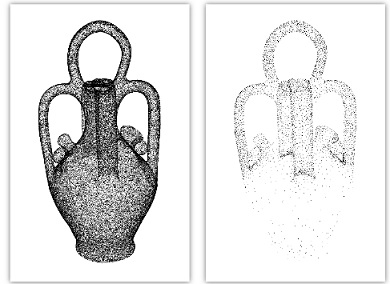SimBa: An efficient tool for approximating Rips-filtration persistence via Simplicial Batch-collapse T. K. Dey, D. Shi, and Y. Wang. European Symposium on Algorithms (ESA 2016). An Extended Version [talk slides] SimBa Software SimPers Software In topological data analysis, a point cloud data P extracted from a metric space is often analyzed by computing the persistence diagram or barcodes of a sequence of Rips complexes built on P indexed by a scale parameter. Unfortunately, even for input of moderate size, the size of the Rips complex may become prohibitively large as the scale parameter increases. Starting with the \emph{Sparse Rips filtration} introduced by Sheehy, some existing methods aim to reduce the size of the complex so as to improve the time efficiency as well. However, as we demonstrate, existing approaches still fall short of scaling well, especially for high dimensional data. In this paper, we investigate the advantages and limitations of existing approaches. Based on insights gained from the experiments, we propose an efficient new algorithm, called SimBa, for approximating the persistent homology of Rips filtrations with quality guarantees. Our new algorithm leverages a batch collapse strategy as well as a new sparse Rips-like filtration. We experiment on a variety of low and high dimensional data sets. We show that our strategy presents a significant size reduction, and our algorithm for approximating Rips filtration persistence is order of magnitude faster than existing methods in practice.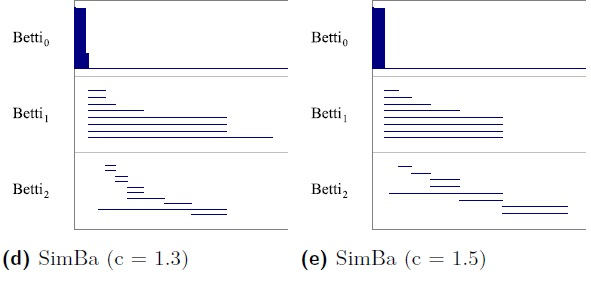Multiscale Mapper: Topological summarization via codomain covers T. K. Dey, F. Memoli, and Y. Wang. ACM-SIAM Sympos. Discrete Algorithms (SODA 2016)  Older version arXiv: 1504.03763v1 [talk slides] SODA version Summarizing topological information from datasets and maps defined on them is a central theme in topological data analysis. Mapper, a tool for such summarization, takes as input both a possibly high dimensional dataset and a map defined on the data, and produces a summary of the data by using a cover of the codomain of the map. This cover, via a pullback operation to the domain, produces a simplicial complex connecting the data points. The resulting view of the data through a cover of the codomain offers flexibility in analyzing the data. However, it offers only a view at a fixed scale at which the cover is constructed. Inspired by the concept, we explore a notion of hierarchical family of coverings which induces a hierarchical family of simplicial complexes connected by simplicial maps, which we call multiscale mapper. We study the resulting structure, its associated persistence module, and its stability under perturbations of the maps and the coverings. The information encoded in multiscale mapper complements that of individual mappers at fixed scales. An upshot of this development is a practical algorithm for computing the persistence diagram of multiscale mapper when the domain is a simplicial complex and the map is a real-valued piecewise-linear function.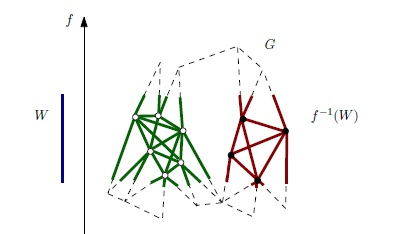Segmenting a surface mesh into pants using Morse theory M. Hajij, T. K. Dey, and X. Li. Graphical Models, Vol 88 (2016), 12--21. http://www.sciencedirect.com/science/article/pii/S1524070316300376 A pair of pants is a genus zero orientable surface with three boundary components. A pants decomposition of a surface is a finite collection of unordered pairwise disjoint simple closed curves embedded in the surface that decompose the surface into pants. In this paper, we present two Morse theory based algorithms for pants decomposition of a surface mesh. Both algorithms operate on achoice of an appropriate Morse function on the surface. The first algorithm uses this Morse function to identify handles that are glued systematically to obtain a pants decomposition. The second algorithm uses the Reeb graph of the Morse function to obtain a pants decomposition. Both algorithms work for surfaces with or without boundaries. Our perliminary implementation of the two algorithms shows that both algorithms run in much less time than an existing state-of-the-art method, and the Reeb graph based algorithm achieves the best time efficiency. Finally, we demonstrate the robustness of our algorothms agaisnt noise.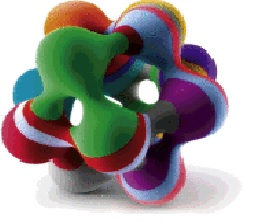Comparing graphs via persistence distortion T. K. Dey, D. Shi and Y. Wang. 31st Annu. Sympos. Comput. Geom. (SoCG 15). [GraphComp software] Metric graphs are ubiquitous in science and engineering. For example, many data are drawn from hidden spaces that are graph-like, such as the cosmic web. A metric graph offers one of the simplest yet still meaningful ways to represent the non-linear structure hidden behind the data. In this paper, we propose a new distance between two finite metric graphs, called the persistence distortion-distance, which draws upon a topological idea. This topological perspective along with the metric space viewpoint provide a new angle to the graph matching problem. Our persistence-distortion distance has two properties not shared by previous methods: First, it is stable against the perturbations of the input graph metrics. Second, it is a \emph{continuous} distance measure, in the sense that it is defined on an alignment of the underlying spaces of input graphs, instead of merely their nodes. This makes our persistence-distortion distance robust against, for example, different discretizations of the same underlying graph. Despite considering the input graphs as continuous spaces, that is, taking all points into account, we show that we can compute the persistence-distortion distance in polynomial time. The time complexity for the discrete case where only graph nodes are considered is much faster. We also provide some preliminary experimental results to demonstrate the use of the new distance measure.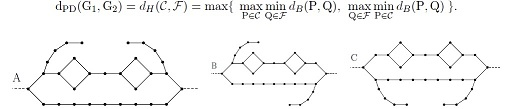M. Buchet,  F. Chazal, T. K. Dey, F. Fan, S. Oudot and Y. Wang. 31st Annu. Sympos. Comput. Geom. (SoCG 15). arXiv:1412.1680. Given a real-valued function ff  defined over a manifold MM  embedded in R^dRd, we are interested in recovering structural information about f f from the sole information of its values on a finite sample PP. Existing methods provide approximation to the persistence diagram of ff  when the noise is bounded in both the functional and geometric domains. However, they fail in the presence of aberrant values, also called outliers, both in theory and practice. We propose a new algorithm that deals with outliers. We handle aberrant functional values with a method inspired from the k-nearest neighbors regression and the local median filtering, while the geometric outliers are handled using the distance to a measure. Combined with topological results on nested filtrations, our algorithm performs robust topological analysis of scalar fields in a wider range of noise models than handled by current methods. We provide theoretical guarantees on the quality of our approximation and some experimental results illustrating its behavior.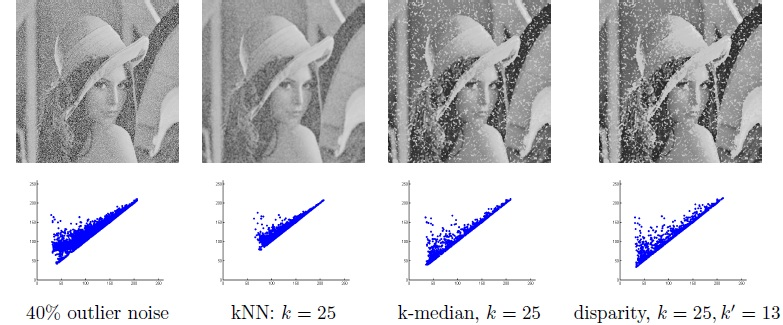Spectral concentration and greedy k-clustering T. K. Dey,  A. Rossi., and A. Sidiropoulos,  arXiv:1404.1008v2. Comput. Geom. Theory & Applications (2018), to appear. A popular graph clustering method is to consider the embedding of an input graph into R^k induced by the first k eigenvectors of its Laplacian, and to partition the graph via geometric manipulations on the resulting metric space. Despite the practical success of this methodology, there is limited understanding of several heuristics that follow this framework. We provide theoretical justification for one such natural and computationally efficient variant. Our result can be summarized as follows. A partition of a graph is called strong if each cluster has small external conductance, and large internal conductance. We present a simple greedy spectral clustering algorithm which returns a partition that is provably close to a suitably strong partition, provided that such a partition exists. A recent result shows that strong partitions exist for graphs with a sufficiently large spectral gap between the k-th and (k+1)-th eigenvalues. Taking this together with our main theorem gives a spectral algorithm which finds a partition close to a strong one for graphs with large enough spectral gap. We also show how this simple greedy algorithm can be implemented in near-linear time for any fixed k and error guarantee. Finally, we evaluate our algorithm on some real-world and synthetic inputs.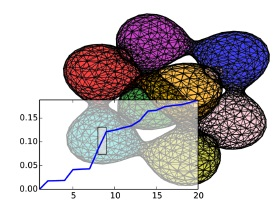Dimenison detection with local homology T. K. Dey,  F. Fan, and Y. Wang, Canadian Conf. Comput. Geom. (CCCG 2014), Full version  arXiv: 1405.3534 [Talk Slide] Detecting the dimension of a hidden manifold from a point sample has become an important problem in the current data-driven era. Indeed, estimating the shape dimension is often the first step in studying the processes or phenomena  associated to the data. Among the many dimension detection algorithms proposed in various fields, a few can provide theoretical guarantee on the correctness of the estimated dimension. However, the correctness usually requires certain regularity of the input: the input points are either uniformly randomly sampled in a statistical setting, or they form the so-called $(\eps,\delta)$-sample which can be neither too dense nor too sparse. Here, we propose a purely topological technique to detect dimensions. Our algorithm is provably correct and works under a more relaxed sampling condition: we do not require uniformity, and we also allow Hausdorff noise. Our approach detects dimension by determining local homology. The computation of this topological structure is much less sensitive to the local distribution of points, which leads to the relaxation of the sampling conditions. Furthermore, by leveraging various developments in computational topology, we show that this local homology at a point $z$ can be computed \emph{exactly} for manifolds using Vietoris-Rips complexes whose vertices are confined within a local neighborhood of $z$. We implement our algorithm and demonstrate the accuracy and robustness of our method using both synthetic and real data sets.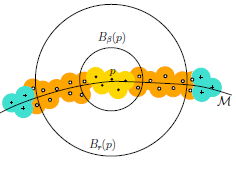Computing topological persistence for simplicial maps T. K. Dey,  F. Fan, and Y. Wang., (SoCG 2014), Proc. 30th Annu. Sympos. Comput. Geom. (2013).  (our algorithm works for any finite field--see the conclusion of updated Full version) [SimpPers software] [Talk slide] Algorithms for persistent homology and zigzag persistent homology are well-studied for homology modules where homomorphisms are induced by inclusion maps. In this paper, we propose a practical algorithm for computing persistence under Z_2 coefficients for a sequence of general simplicial maps. First, we observe that it is not hard to simulate simplicial maps by inclusion maps but not necessarily in a monotone direction. This, combined with the known algorithms for zigzag persistence, provides an algorithm for computing the persistence induced by simplicial maps. Our main result is that the above simple minded approach can be improved for a sequence of simplicial maps given in a monotone direction. The improvement results from the use of the so-called annotations that we show can determine the persistence of simplicial maps using a lighter data structure. A consistent annotation through atomic operations implies the maintenance of a consistent cohomology basis, hence a homology basis by duality. While the idea of maintaining a cohomology basis through an inclusion is not new, maintaining them through a vertex collapse is new, which constitutes an important atomic operation for simulating simplicial maps. Annotations support the vertex collapse in addition to the usual inclusion quite naturally. Finally, we exhibit an application of this new tool in which we approximate the persistence diagram of a filtration of Rips complexes where vertex collapses are used to tame the blow-up in size.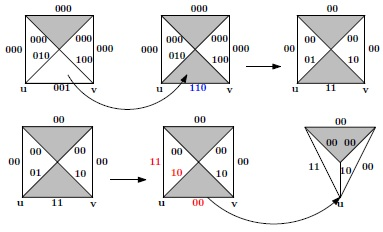Automatic posing of meshed human model using point clouds T. K. Dey,  B. Fu, H. Wang, and L. Wang., (SMI 2014), Computers & Graphics, Vol. 46, pages 14--24. We introduce a markerless approach to deform a quality human body template mesh from its original pose to a different pose specified by a point cloud. The point cloud may be noisy, incomplete, or even captured from a different person. In this approach, we first build coarse correspondences between the template mesh and the point cloud through a squeezed spectral embedding technique that exploits human body extremities. Based on these correspondences, we define the goal of non-rigid registration using an elastic energy functional and apply a discrete gradient flow to reduce the difference between a coarse control mesh and the point cloud. The deformed template mesh can then be obtained from the deformation of the control mesh using mean value coordinates afterwards. Our experiments show (see the supplementary video) that the approach is capable of equipping a mesh with the pose of a scanned point cloud data even if it is incomplete and noisy.Edge contractions and simplicial homology T. K. Dey,  A. N. Hir ani, B. Krishnamoorthy, and G. Smith. April, 2013. arXiv:1304.0664 We study the effect of edge contractions on simplicial  homology because these contractions have turned to be useful in various  applications involving topology. It was observed previously that   contracting edges that  satisfy the so called  link condition preserves homeomorphism  in low dimensional complexes, and homotopy in general.   But, checking the link condition involves computation in all dimensions,  and hence can be costly, especially in high dimensional  complexes. We define a weaker and more local condition called the p-link condition for each dimension p, and study its  effect on edge contractions. We prove the following: (i) For homology  groups, edges satisfying the  p- and (p-1)-link conditions can be contracted without  disturbing the p-dimensional homology group. (ii) For  relative homology groups, the (p-1)-, and the (p-2)-link conditions  suffice to guarantee that the contraction does not introduce any new class in  any of the resulting relative  homology groups, though some of the existing classes can be  destroyed. Unfortunately, the surjection in relative homolgy groups  does not guarantee that no new relative  torsion is created. (iii) For torsions, edges satisfying the p-link condition alone can be contracted without creating any new relative torsion and the p-link condition cannot be avoided.  The  results on relative homology and relative torsion are motivated by  recent results on computing optimal homologous chains, which state  that such problems can be solved by linear programming if the  complex has no relative torsion.  Edge contractions that do not  introduce new relative torsions, can safely be availed in these contexts.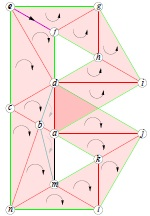Graph induced complex on point data (full version) T. K. Dey,  F. Fan, and Y. Wang., (SoCG 2013) Proc. 29th Annu. Sympos. Comput. Geom. (2013), pages 107--116. [Talk slides] [Web-page] [GICsoftware] The efficiency of extracting topological information from point data depends largely on the complex that is built on top of the data points. From a computational viewpoint, the most favored complexes for this purpose have so far been Vietoris-Rips and witness complexes. While the Vietoris-Rips complex is simple to compute and is a good vehicle for extracting topology of sampled spaces, its size is huge--particularly in high dimensions. The witness complex on the other hand enjoys a smaller size because of a subsampling, but fails to capture the topology in high dimensions unless imposed with extra structures. We investigate a complex called the graph induced complex that, to some extent, enjoys the advantages of both. It works on a subsample but still retains the power of capturing the topology as the Vietoris-Rips complex. It only needs a graph connecting the original sample points from which it builds a complex on the subsample thus taming the size considerably. We show that, using the graph induced complex one can (i) infer the one dimensional homology of a manifold from a very lean subsample, (ii) reconstruct a surface in three dimension from a sparse subsample without computing Delaunay triangulations, (iii) infer the persistent homology groups of compact sets from a sufficiently dense sample. We provide experimental evidences in support of our theory.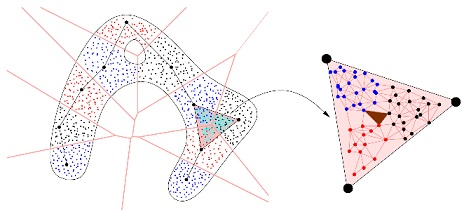The compressed annotation matrix: an efficient data structure for computing persistent cohomology.J.-D. Boissonnat, T. K. Dey,  C. Maria, (ESA 2013), LNCS 8125, 2013. Persistent homology with coefficients in a field F coincides with the same for cohomology because of duality. We propose an implementation of a recently introduced algorithm for persistent cohomology that attaches annotation vectors with the simplices. We separate the representation of the simplicial complex from the representation of the cohomology groups, and introduce a new data structure for maintaining the annotation matrix, which is more compact and reduces substancially the amount of matrix operations. In addition, we propose a heuristic to simplify further the representation of the cohomology groups and improve both time and space complexities. The paper provides a theoretical analysis,  as well as a detailed experimental study of our implementation and comparison with state-of-the-art software for persistent homology and cohomology. Note: Also see our computing topological persistence for simplicial maps" paper which motivated this implementation.
 An efficient computation of handle and tunnel loops via Reeb graphs. T. K. Dey,  F. Fan, and Y. Wang. ACM Trans. Graphics (Siggraph 2013), Vol. 32 (4). Supplementary file for proofs.A special family of non-trivial loops on a surface called handle and tunnel loops associates closely to geometric features of handles" and tunnels" respectively in a 3D model. The identification of these handle and tunnel loops can benefit a broad range of applications from topology simplification / repair, and surface parameterization, to feature and shape recognition. Many of the existing efficient algorithms for computing non-trivial loops cannot be used to compute these special type of loops. The two algorithms known for computing handle and tunnel loops provably have a serious drawback that they both require a tessellation of the interior and exterior spaces bounded by the surface. Computing such a tessellation of three dimensional space around the surface is a non-trivial task and can be quite expensive. Furthermore, such a tessellation may need to refine the surface mesh, thus causing the undesirable side-effect of outputting the loops on an altered surface mesh. In this paper, we present an efficient algorithm to compute a basis for handle and tunnel loops without requiring any 3D tessellation. This saves time considerably for large meshes making the algorithm scalable while computing the loops on the original input mesh and not on some refined version of it. We use the concept of the Reeb graph which together with several key theoretical insights on linking number provide an initial set of loops that provably constitute a handle and a tunnel basis. We further develop a novel strategy to tighten these handle and tunnel basis loops to make them geometrically relevant. We demonstrate the efficiency and effectiveness of our algorithm as well as show its robustness against noise, and other anomalies in the input.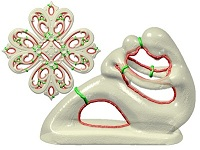Localized Delaunay Refinement for Piecewise-Smooth Complexes (full version) T. K. Dey and A. Slatton  (SoCG 2013) Proc. 29th Annu. Sympos. Comput. Geom. (2013), pages 47--56. [software LocPSC] [Talk Slides] The Delaunay refinement, a versatile method of mesh generation, is plagued by memory thrashing when required to generate large output meshes. To address this space issue, a localized version of Delaunay refinement was proposed for generating meshes for smooth surfaces and volumes bounded by them. The method embodies a divide-and-conquer paradigm in that it maintains the growing set of sample points with an octree and produces a local mesh within each individual node, and stitches these local meshes seamlessly. The proofs of termination and global consistency for localized methods exploit recently developed sampling theory for smooth surfaces. Unfortunately, these proofs break down for a larger class called piecewise smooth complexes (PSCs) that allow smooth surface patches that are joined along ridges and corners. In this work, we adapt a recently developed sampling and meshing algorithm for PSCs into the localization framework. This requires revisiting the original algorithm, and more importantly re-establishing the correctness proofs to accommodate the localization framework. Our implementation of the algorithm exhibits that it can indeed generate large meshes with significantly less time and memory than the original algorithm without localization. In fact, it beats a state-of-the-art meshing tool of CGAL for generating large meshes.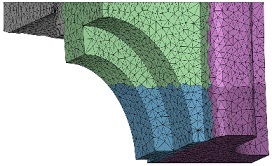Voronoi-based Feature Curves Extraction for Sampled Singular Surfaces T. K. Dey and L. Wang  (SMI 2013), Computers & Graphics, special issue of Shape Modeling International (2013), Vol. 37 (6), 659--668. [Web-page] [software SingularCocone] The detection and reconstruction of feature curves in surfaces from a point cloud data is a challenging problem because most of the known theories for smooth surfaces break down at these places. The features such as boundaries, sharp ridges and corners, and curves where multiple surface patches intersect creating non-manifold points are often considered important geometries for further processing. As a result, they need to be preserved in a reconstruction of the sampled surface from its point sample. The problem becomes harder in presence of noise. We propose a robust Voronoi-based pipeline that engages several sub steps consisting of approaches proposed originally for smooth case. We modify or enhance them to handle features in singular surfaces. The experimental results provide the evidence that the method is effective. Note: This paper extracts features differently than our Feature-Preserving reconstruction of singular surfaces" paper which introduced the WeightCocone to reconstruct with feature curves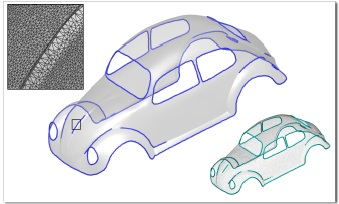Weighted graph Laplace operator under topological noise T. K. Dey, P. Ranjan, and Y. Wang. (SODA 2013), ACM-SIAM Symposium on Discrete Algorithms (2013). Recently, various applications have motivated the study of spectral structures (eigenvalues and eigenfunctions) of the so-called Laplace-Beltrami operator of a manifold and their discrete versions. A popular choice for the discrete version is the so-called Gaussian weighted graph Laplacian which can be applied to point cloud data that samples a manifold. Naturally, the question of stability of the spectrum of this discrete Laplacian under the perturbation of the sampled manifold becomes important for its practical usage. Previous results showed that the spectra of both the manifold Laplacian and discrete Laplacian are stable when the perturbation is nice'' in the sense that it is restricted to a diffeomorphism with minor area distortion. However, this forbids, for example, small topological changes. We study the stability of the spectrum of the weighted graph Laplacian under more general perturbations. In particular, we allow arbitrary, including topological, changes to the hidden manifold as long as they are localized in the ambient space and the area distortion is small. Manifold Laplacians may change dramatically in this case. Nevertheless, we show that the weighted graph Laplacians computed from two sets of points, uniformly randomly sampled from a manifold and a perturbed version of it, have similar spectra. The distance between the two spectra can be bounded in terms of the size of the perturbation and some intrinsic properties of the original manifold.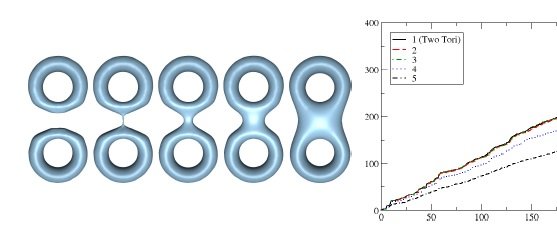Animating bubble interactions in a liquid foam O. Busaryev, T. K. Dey, H. Wang, and R. Zhong. (SIGGRAPH 2012), ACM Trans. Graphics, Vol. 31 (4), 2012. [Video link] Bubbles and foams are important features of liquid surface phenomena, but they are difficult to animate due to their thin films and complex interactions in the real world. In particular, small bubbles (having diameter <1cm) in a dense foam are highly affected by surface tension, so their shapes are much less deformable compared with larger bubbles. Under this small bubble assumption, we propose a more accurate and efficient particle-based algorithm to simulate bubble dynamics and interactions. The key component of this algorithm is an approximation of foam geometry, by treating bubble particles as the sites of a weighted Voronoi diagram. The connectivity information provided by the Voronoi diagram allows us to accurately model various interaction effects among bubbles. Using Voronoi cells and weights, we can also explicitly address the volume loss issue in foam simulation, which is a common problem in previous approaches. Under this framework, we present a set of bubble interaction forces to handle miscellaneous foam behaviors, including foam structure under Plateau's laws, clusters formed by liquid surface bubbles, bubble-liquid and bubble-solid coupling, bursting and coalescing. Our experiment shows that this method can be straightforwardly incorporated into existing liquid simulators, and it can efficiently generate realistic foam animations, some of which have never been produced in graphics before.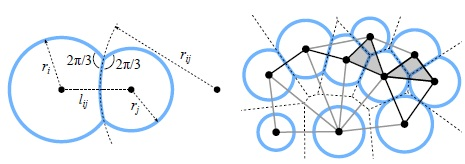Feature-Preserving reconstruction of singular surfaces T. K. Dey, X. Ge, Q. Que, I. Safa, L. Wang, Y. Wang. Computer Graphics Forum, Vol. 31 (5), 1787--1796, special issue of Eurographics Sympos. Geometry Processing (SGP 2012). [Talk slides] Reconstructing a surface mesh from a set of discrete point samples is a fundamental problem in geometric modeling. It becomes challenging in presence of singularities' such as boundaries, sharp features, and non-manifolds. A few of the current research in reconstruction have addressed handling some of these singularities, but a unified approach to handle them all is missing. In this paper we allow the presence of various singularities by requiring that the sampled object is a collection of smooth surface patches with boundaries that can meet orintersect. Our algorithm first identifies and reconstructs the features where singularities occur. Next, it reconstructs the surface patches containing these feature curves. The identification and reconstruction of feature curves are achieved by a novel combination of the Gaussian weighted graph Laplacian and the Reeb graphs. The global reconstruction is achieved by a method akin to the well known Cocone reconstruction, but with weighted Delaunay triangulation that allows protecting the feature samples with balls. We provide various experimental results to demonstrate the effectiveness of our feature-preserving singular surface reconstruction algorithm.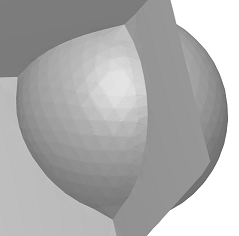Topological Persistence for circle valued maps D. Burghelea and T. K. Dey. Discrete & Computational Geometry, Volume 50, No. 1 (2013), 69--98.  Also in  arXiv:1104.5646. April, 2011. We study circle valued maps and consider the persistence  of the homology of their fibers. The outcome is a finite collection of computable invariants  which answer the basic questions on persistence and in addition encode the topology of the source space and its relevant subspaces. Unlike persistence of real valued maps, circle valued maps enjoy a different class of invariants celled Jordan cells in addition to bar codes. We establish a relation between the homology of the source space and of its relevant subspaces with these invariants and provide a new algorithm to compute these invariants from an input matrix that encodes a circle valued map on an input simplicial complex. Also see how it all started: arXiv:1012.3763, 2010.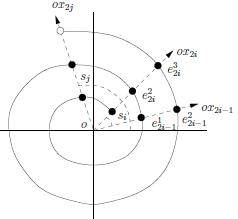Eigen deformation of 3D models T. K. Dey, P. Ranjan and Y. Wang. Proc. Computer Graphics International (CGI 2012). The Visual Computer Volume 28, Numbers 6-8 (2012), 585-595, DOI: 10.1007/s00371-012-0705-0  [Talk slides] [Video] Recent advances in mesh deformation have been dominated by two techniques: one uses an interactive structure like a cage which transfers the user intended moves to the mesh, the other lest the user to impart the moves to the mesh directly. The former one lets the user deform the model in real-time and also preserves the shape with sophisticated techniques like Green Coordinates. The direct techniques on the other hand free the user from the burden of creating an appropriate cage though they take more computing time to solve larger non-linear optimizations. It would be ideal to develop a cage-free technique that provides real-time defoirmation while respecting the local geometry. Using a simple eigen-framework we devise such a technique. Our framework creates an implicit skeleton automatically. The user only specifies the motion in a simple and intuitive manner, and our algorithm computes a deformation whose quality is similar to that of the cage-based scheme with Green Coordinates.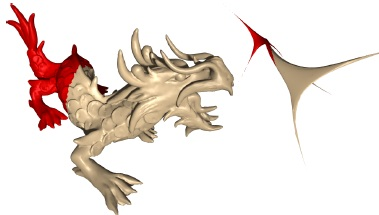Annotating simplices with a homology basis and its applications O. Busaryev, S. Cabello, C. Chen, T. K. Dey, and Y. Wang. 13th Scandinavian Sympos. Workshops Algorithm Theory (SWAT 2012). Lecture Notes in Computer Science Volume 7357, 2012, pp 189-200.  [Talk slides] Earlier arxiv version arXiv:1107.3793v2 Let K be a simplicial complex and g the dimension of its p-th homology group H_p(K) defined with Z_2 coefficients. We show that we can compute a basis H of H_p(K) and annotate each p-simplex of K with a binary vector of length g with the following property: the annotations, summed over all p-simplices in any p-cycle z, provide the coordinate vector of the homology class [z] in the basis H. The basis and the annotations for all simplices can be computed in O(n^{\omega}) time, where n is the size of K and \omega<2.376 is a quantity so that two nxn  matrices can be multiplied in O(n^{\omega}) time. The pre-computation of annotations permits answering queries about the independence or the triviality of p-cycles efficiently. Using annotations of edges in 2-complexes, we derive better algorithms for computing optimal basis and optimal homologous cycles in 1-dimensional homology. Specifically, for computing an optimal basis of H_1(K),we improve the time complexity known for the problem from O(n^4) to O(n^{\omega}+n^2g^{\omega-1}). Here n denotes the size of the 2-skeleton of K and g the rank of H_1(K). Computing an optimal cycle homologous to a given 1-cycle is NP-hard even for surfaces and an algorithm taking 2^{O(g)}n\log n time is known for surfaces. We extend this algorithm to work with arbitrary 2-complexes in O(n^{\omega})+2^{O(g)}n^2\log n time using annotations. Also see this technical report for a more practical algorithm.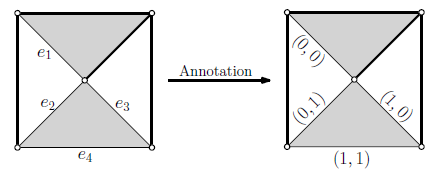Localized Delaunay refinement for volumes T. K. Dey and A. G. Slatton.  Computer Graphics Forum, Vol 30 (5), 1417--1426. Special issue Proc. of Eurographics Sympos. Geometry Processing (SGP 2011). [Web-page] [Talk slides] [Software] Delaunay refinement, recognized as a versatile tool for meshing a variety of geometries, has the deficiency that it does not scale well with increasing mesh size. The bottleneck can be traced down to the memory usage of 3D Delaunay triangulations. Recently an approach has been suggested to tackle this problem for the specific case of smooth surfaces by subdividing the sample set in an octree and then refining each subset individually while ensuring {\em termination} and {\em consistency}. We extend this to localized refinement of volumes, which brings about some new challenges. We show how these challenges can be met with simple steps while retaining provable guarantees, and that our algorithm scales many folds better than a state-of-the-art meshing tool provided by CGAL. Also, see the paper below on localized Delaunay refinement for surfaces.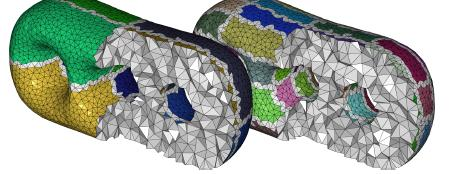Reeb graphs: approximation and persistence T. K. Dey and Y. Wang.  Proc. 27th Annu. Sympos. Comput. Geom. (SOCG 2011), 226--235.  Extended version in Discrete & Computational Geometry, Vol. 49 (2013),  46--73. [Extended version] Given a continuous function f: X-> R on a topological space X, its {\em level set} f^{-1}(a) changes continuously as the real value a changes. Consequently, the connected components in the level sets appear, disappear, split and merge.  The Reeb graph of f summarizes this information into a graph structure. Previous work on Reeb graph mainly focused on its efficient computation. In this paper, we initiate the study of two important aspects of the Reeb graph which can facilitate its  broader applications in shape and data analysis. The first one is the approximation of the Reeb graph of a function on a smooth compact manifold M without boundary. The approximation is computed from a set of points P sampled from M. By leveraging a relation between the Reeb graph and the so-called {\em vertical homology group}, as well as between cycles in M and in a Rips complex  constructed from P, we compute the H_1-homology of the Reeb graph from P. It takes O(n \log n) expected time, where n is the size of the 2-skeleton of the Rips complex. As a by-product, when M is an orientable 2-manifold, we also obtain an efficient near-linear time (expected) algorithm to compute the rank of H_1(M) from point data. The best known previous algorithm for this problem takes O(n^3) time for point data. The second aspect concerns the definition and computation of the \emph{persistent Reeb graph homology} for a sequence of Reeb graphs defined on a filtered space. For a piecewise-linear function defined on a filtration of a simplicial complex K, our algorithm computes all persistent H_1-homology for the Reeb graphs in O(n n_e^3) time, where n is the size of the 2-skeleton and n_e is the number of edges in K.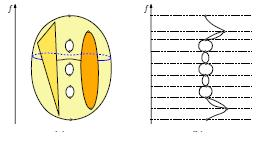Localized Delaunay refinement for sampling and meshing.  [Talk slides][Web-page][Software] T. K. Dey, J. A. Levine, and A. Slatton.  Computer Graphics Forum. Vol. 29 (5)(2010), 1723--1732.  Specail issue of Proc. Eurographics Sympos. Geometry Processing. (SGP 2010). [Extended version] The technique of Delaunay refinement has been recognized as a versatile tool to generate Delaunay meshes of a variety of geometries. Despite its usefulness, it suffers from one lacuna that limits its application. It does not scale well with the mesh size. As the sample point set grows, the Delaunay triangulation starts stressing the available memory space which ultimately stalls any effective progress. A natural solution to the problem is to maintain the point set in clusters and run the refinement on each individual cluster. However, this needs a careful point insertion strategy and a balanced coordination among the neighboring clusters to ensure consistency across individual meshes. We design an octtree based localized Delaunay refinement method for meshing surfaces in three dimensions which meets these goals. We prove that the algorithm terminates and provide guarantees about structural properties of the output mesh. Experimental results show that the method can avoid memory thrashing while computing large meshes and thus scales much better than the standard Delaunay refinement method.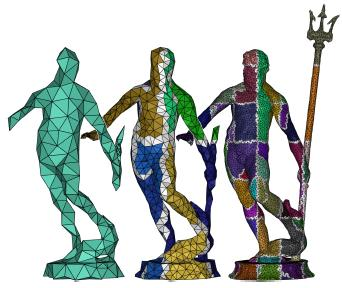Persistent heat signature for pose-oblivious matching of incomplete models.  [Talk slides] T. K. Dey, K. Li, C. Luo, P. Ranjan, I. Safa, and Y. Wang.  Computer Graphics Forum. Vol. 29 (5) (2010), 1545--1554. Special isue of  Proc. Sympos. Geometry Processing. (SGP 2010). Although understanding of shape features in the context of shape matching and retrieval has made considerable progress in recent years, the case for partial and incomplete models in presence of pose variations still begs a robust and efficient solution. A signature that encodes features at multi-scales in a pose invariant manner is more appropriate for this case. The Heat Kernel Signature function from spectral theory exhibits this multi-scale property. We show how this concept can be merged with the persistent homology to design a novel efficient pose-oblivious matching algorithm for all models, be they partial, incomplete, or complete. We make the algorithm scalable so that it can handle large data sets. Several test results show the robustness of our approach.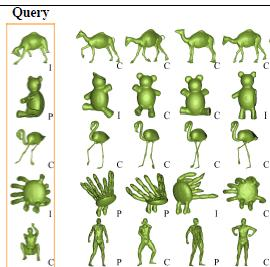Tracking a generator by persistence. O. Busaryev, T. K. Dey, and Y. Wang.   16th Annu. Internat. Computating and Combinatorics Conf. (COCOON 2010), 278--287.  Journal version in Discrete Mathematics, Algorithms and Applications, Vol 2, Issue 4, 2010, 539--552. DOI:10.1142/S1793830910000875. The persistent homology provides a mathematical tool to describe features" in a principle manner. The persistence algorithm proposed by Edelsbrunner et al.  can compute not only the persistent homology for a filtered simplicial complex, but also representative generating cycles for persistent homology groups. However, if there are dynamic changes either in the filtration or in the underlying simplicial complex, the representative generating cycle can change wildly. In this paper, we consider the problem of tracking generating cycles with temporal coherence. Specifically, our goal is to track a chosen essential generating cycle so that the changes in it are local". This requires reordering simplices in the filtration, To handle reordering operations. we build upon the matrix framework proposed by Cohen-Steiner et al. to swap two consecutive simplices, so that we can process a reordering directly. We present an application showing how our algorithm can track an essential cycle in a complex constructed out of a point cloud data.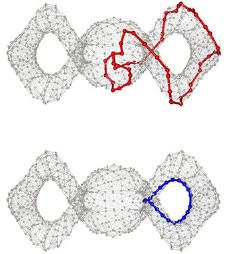Optimal homologous cycles, total unimodularity, and linear programming. T. K. Dey, A. Hirani, and B. Krishnamoorthy.   SIAM J. Computing, Vol. 40, pp 1026--1044. Prelim. version in 42nd ACM Sympos. Comput. Theory (STOC 2010), 221--230. arXiv:1001.0338v1[math.AT], 2nd January, 2010. [web-page] [combined talk-slides] Given a simplicial complex with weights on its simplices, and a nontrivial cycle on it, we are interested in finding the cycle with minimal weight which is homologous to the given one. Assuming that the homology is defined with integer (Z) coefficients, we show the following (Theorem 5.2): For a finite simplicial complex K of dimension greater than p, the boundary matrix [d_(p+1)] is totally unimodular if and only if H_p(L,L_0) is torsion-free for all pure subcomplexes L_0, L in K of dimension p and p+1 respectively where L_0\subset L. Because of the total unimodularity of the boundary matrix, we can solve the optimization problem, which is inherently an integer programming problem, as a linear program and obtain integer solution. Thus the problem of finding optimal cycles in a given homology class can be solved in polynomial time. This result is surprising in the backdrop of a recent result which says that the problem is NP-hard under Z_2 coefficients which, being a field, is in general easier to deal with. One consequence of our result, among others, is that one can compute in polynomial time an optimal 2-cycle in a given homology class for any finite simplicial complex embedded in R^3. Our optimization approach can also be used for various related problems, such as finding an optimal chain homologous to a given one when they are not cycles.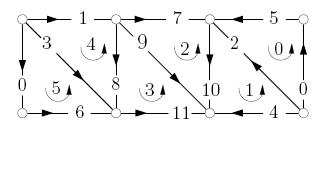Approximating cycles  in a shortest basis of the first homology group from point data T. K. Dey, J. Sun, and Y. Wang.   Inverse Problems, Vol. 27 (2011), 124004. doi:10.1088/0266-5611/27/12/124004 An earlier version appeared with title Approximating loops in a shortest homology basis from point data" in Proc. 26th Annu. Sympos. Comput. Geom. (SOCG 2010), 166--175. arXiv:0909.5654v1[cs.CG], 30th September 2009.  [web-page]  [software] [talk-slides] Inference of topological and geometric attributes of a hidden manifold from its point data is a fundamental problem arising in many scientific studies and engineering applications. In this paper we present an algorithm to compute a set of cycles from a point data that presumably sample a smooth manifold M\subset R^d. These cycles approximate a shortest basis of the one dimensional homology group H_1(M) over coefficients in finite field Z_2.  Previous results addressed the issue of computing the rank of the homology groups from point data, but there is no result on approximating the shortest basis of a manifold from its point sample. In arriving our result, we also present a polynomial time algorithm for computing a shortest basis of H_1(K) for any finite simplicial complex K whose edges have non-negative weights.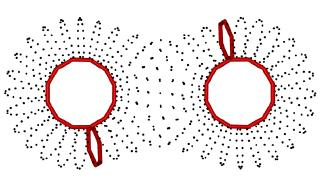Convergence, Stability, and Discrete Approximation of Laplace Spectra T. K. Dey, P. Ranjan, and Y. Wang.   Proc. ACM/SIAM Sympos. Discrete Algorithms (SODA 2010), 650--663. Paper with a minor correction. Spectral methods have been widely used in a broad range of applications fields. One important object involved in such methods is the Laplace-Beltrami operator of a manifold. Indeed, a variety of work in graphics and geometric optimization uses the eigen-structures (i.e., the eigenvalues and eigenfunctions) of the Laplace operator. Applications include mesh smoothing, compression, editing, shape segmentation, matching, parameterization, and so on. While the Laplace operator is defined (mathematically) for a smooth domain, these applications often approximate a smooth manifold by a discrete mesh. The spectral structure of the manifold Laplcian is estimated from some discrete Laplace operator constructed from this mesh. In this paper, we study the important question of how well the specturm computed from the discrete mesh approximates the true spectrum of the manifold Lplacian. We exploit a recent result on mesh Laplacian and provide the first convergence result to relate the spectrum constructed from a general mesh (approximating an m-manifold embedded in R^d) with the true spectrum. We also study how stable these eigenvalues and their discrete approximations are when the underlying manifold is perturbed, and provide explicit bounds for the Laplacian spectra of two close" manifolds, as well as a convergence result for their discrete approximations. Finally, we present various experimental results to demonstrate that these discrete spectra are both accurate and robust in practice.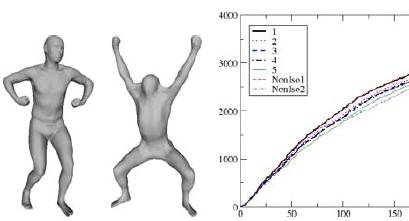Repairing and meshing imperfect shapes with Delaunay refinement O. Busaryev, T. K. Dey, J. A. Levine.   Proc.  SIAM/ACM Joint Conf. Geometric and Physical Modeling (SPM 2009), 25--33. As a direct consequence of software quirks, designer errors, and representation flaws, often three-dimensional shapes are stored in formats that introduce inconsistencies such as small gaps and overlaps between surface patches. We present a new algorithm that simultaneously repairs imperfect geometry and topology while generating Delaunay meshes of these shapes. At the core of this approach is a meshing algorithm for input shapes that are piecewise smooth complexes (PSCs), a collection of smooth surface patches meeting at curves non-smoothly or in  non-manifold configuarations. Guided by a user tolerance parameter, we automatically merge nearby components while building a Delaunay mesh that has many of these errors fixed. Experimental evidence is provided to show the results of our algorithm on common computer-aided design (CAD) formats. Our algorithm may  also be used to simplify shapes by removing small features which would require an excessive number of elements to preserve them in the output mesh. Note: One of the main ingredients of this paper is our PSC meshing paper.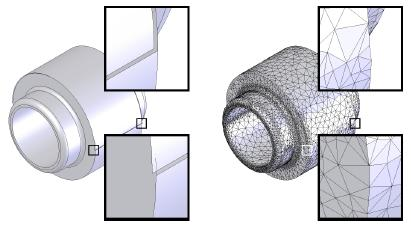Isotopic Reconstruction of Surfaces with Boundaries T. K. Dey, K. Li., E. A. Ramos, and R. Wenger.   Proc.  Sympos. Geom. Processing.(SGP09), special issue of Computer Graphics Forum, Vol. 28, No. 5 (2009), 1371--1382. [Web-page] [Software] [talk-slide] [Video] We present an algorithm for the reconstruction of a surface with boundaries (including a non-orientable one) in three dimensions from a sufficiently dense sample. It is guaranteed that the output is isotopic to the unknown sampled surface. No previously known algorithm guarantees isotopic or homeomorphic reconstruction of surfaces with boundaries. Our algorihtm is surprisingly simple. It peels' slivers greedily from an alpha-complex of a sample of the surface. No other post-processing is necessary. We provide several experimental results from an implementation of our basic algorithm and also a modified version of it.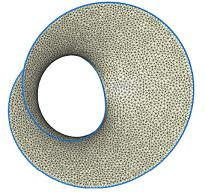Cut Locus and Topology from Surface Point Data. T. K. Dey, K. Li. Proc. 25th Ann. Sympos. Comput. Geom.(SOCG09), 2009, 125--134. A cut locus of a point in a compact Riemannian manifold M is defined as the set of points where minimizing geodesics issued from p stop being minimizing. It is known that a cut locus contains most of the topological information of M. Our goal is to utilize this property of cut loci to decipher the topology of M from a point sample. Recently it has been shown that Rips complexes can be built from  a point sample P of M systematically to compute the Betti numbers, the rank of the homology groups of M. Rips complexes can be computed easily and therefore are favored over others such as restricted Delaunay, alpha, Cech, and witness complex. However, the sizes of the Rips complexes tend to be large. Since the dimension of a cut locus is lower than that of the manifold M, a subsample of P approximating the cut locus is usually much smaller in size and hence admits a relatively smaller Rips complex. In this paper we explore the above approach for point data sampled from surfaces embedded in any high dimensional Euclidean space. We present an algorithm that computes a subsample P' of a sample P of a 2-manifold where P' approximates a cut locus. Empirical results show that the first Betti number of M can be computed from Rips complexes built on these subsamples. The sizes of these Rips complexes are much smaller than the one built on the original sample of M. Our first attempt on a related topic was the following paper: Topology from Data via Geodesic Complexes. T. K. Dey and K. Li. Tech Report OSU-CISRC-3/09-TR05.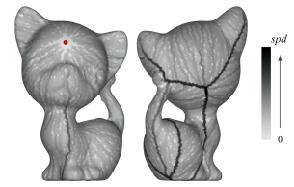Delaunay meshing of piecewise smooth complexes without expensive predicates. T. K. Dey, J. A. Levine.  Algorithms, vol. 2, issue 4 (2009), 1327--1349. doi:10.3390/a2041327 Tech Report, OSU-CISRC-7/08-TR40, July 2008. [Video][Software][Talk-slide][Web-page] Recently a Delaunay refinement algorithm has been proposed that can mesh piecewise smooth complexes which include polyhedra, smooth and piecewise smooth surfaces, and non-manifolds. However, this algorihtm employs domain dependent numerical predicates, some of which could be computationally expensive and hard to implement. In this paper we develop a refinement strategy that eliminates these complicated domain dependent predicates. As a result we obtain a meshing algorithm that is practical and implementation-friendly.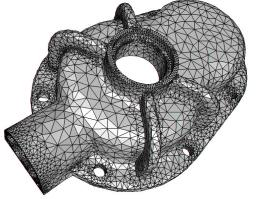Persistence-based Handle and Tunnel Loops Computation Revisited for Speed Up. T. K. Dey and K. Li. Shape Modeling International (SMI09), 2009. Special issue of Computer & Graphics, Article in press, doi:10.1016/j.cag.2009.03.008. [Software] Loops in surfaces associated with topological features such as handles and tunnels are important entities in many applications including surface parameterization, feature identification, and topological simplification. Recently, a persistent homology based algorithm has been proposed to compute them. The algorithm has several advantages including its simplicity, combinatorial nature and independence from computing other extra structures. In this paper, we propose changes to this loop computation algorithm based on some novel observations. These changes reduce the computation time of the algorithm dramatically. In particular, our experimental results show that the suggested changes achieve considerable speed up for large data sets without sacrificing loop qualities. Note: This is a follow-up paper of our SIGGRAPH08 paper below.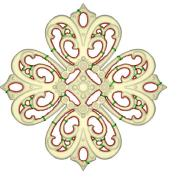Computing Geometry-aware Handle and Tunnel Loops in 3D Models. T. K. Dey, K. Li, J. Sun, and D. Cohen-Steiner. SIGGRAPH 2008, 45:1--45:9. [Video][Software][Talk-slide][Web-page] Many applications such as topology repair, model editing, surface parameterization, and feature recognition benefit from computing loops on surfaces that wrap around their handles' and tunnels'. Computing such loops while optimizing their geometric lengths is difficult. On the other hand, computing such loops without considering geometry is easy but may not be very useful. In this paper we strike a balance by computing topologically correct loops that are also geometrically relevant. Our algorithm is a novel application of the concepts from topological persistence introduced recently in computational topology. The usability of the computed loops is demonstrated with some examples in feature identification and topology simplification. Note: See the paper below  On computing handle and tunnel loops  for our first attempt on this problem.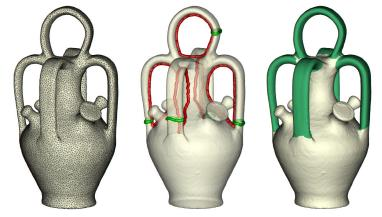Recursive geometry of the flow complex and topology of the flow complex filtration. K. Buchin, T. K. Dey, J. Giesen, and M. John. Comput. Geom. Theory Application. (2008), vol. 40, 115--157. The flow complex is a geometric structure, similar to the Delaunay tessellation, to organize a set of (weighted) points in R^k. Flow shapes are topological spaces correspoding to substructures of the flow complex. The flow complex and flow shapes have found applications in surface reconstruction, shape matching, and molecular modeling. In this article we give an algorithm for computing the flow complex of weighted points in any dimension. The algorithm reflects the recursive structure of the flow complex. On the basis of the algorithm we establish a topological similarity between flow shapes and the nerve of a corresponding ball set, namely homotopy equivalence. This paper grew out of: Alpha-Shapes and Flow Shapes are Homotopy Equivalent. T. K. Dey, J. Giesen and M. John. Proceedings of the 35th Annual ACM Symposium on Theory of Computing (STOC 2003) 493-502
 Normal variation with adaptive feature size (2007) The proof of the normal variation lemma (Lemma 2) in  Amenta-Bern paper Surface reconstruction by Voronoi filtering", DCG, 1999, pg. 481-504, is not correct (which also appears in my book on reconstruction). In this short note we fix the problem and improve the bound on the normal variation. This should improve various bounds derived afterwards using the normal variation result.
 Maintaining Deforming Surface Meshes S.-W. Cheng and T. K. Dey. Proc. 19th ACM-SIAM Sympos. Discrete Algorithms (SODA 2008) (2008), 112--121. We present a method to maintain a mesh approximating a deforming surface, which is specified by a dense set of sample points. We identify a reasonable motion model for which a provably good surface mesh can be maintained. Our algorithm determines the appropriate times at which the mesh is updated to maintain a good approximation. The updates use simple primitives, and no costly computation such as line-surface intersection is necessary. Point insertions and deletions are allowed at the updates. Each update takes time linear in the size of the current sample set plus the new sample points inserted. We also construct examples for which, under the same model, no other algorithm makes asymptotically fewer changes to the mesh than our algorithm. This paper is based on the following flip paper available as a preprint from computational geoemtry arxiv.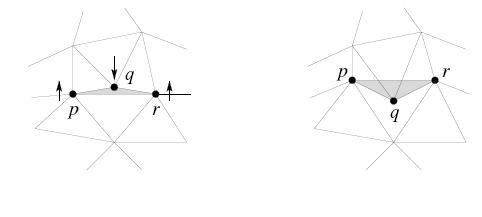Delaunay Edge Flips in Dense Surface Triangulations S.-W. Cheng and T. K. Dey. Pre-print arXiv:cs.CG/0712.1959, 2007. short version in EuroCG 2008. Note: We are retracting this result due to a bug in a Lemma (Lemma 3.1). We are trying to salvage the other results without using Lemma 3.1. The abstract was: Delaunay flip is an elegant, simple tool to convert a triangulation of a point set to its Delaunay triangulation. The technique has been researched extensively for full dimensional triangulations of point sets. However, an important case of triangulations which are not full dimensional is surface triangulations in three dimensions. In this paper we address the question of converting a surface triangulation to a subcomplex of the Delaunay triangulation with edge flips. We show that the surface triangulations which closely approximate a smooth surface with uniform density can be transformed to a Delaunay triangulation with a simple edge flip algorithm. The condition on uniformity becomes less stringent with increasing density of the triangulation. If the condition is dropped completely, the flip algorithm still terminates although the output surface triangulation becomes almost Delaunay" instead of exactly Delaunay.
 A practical Delaunay meshing algorithm for a large class of domains S.-W. Cheng, T. K. Dey, and J. A. Levine.  Proc. 16th  Internat. Meshing Roundtable (IMR 2007), 477--494. [Talk slides] Recently a Delaunay refinement algorithm has been proposed that can mesh domains as general as piecewise smooth complexes [CDR07]. In this paper we introduce a novel modification of the algorithm  to make it implementable in practice. In particular, we replace four tests of the original algorithm with only a single test that is easy to implement. The algorithm has the following guarantees. The output mesh restricted to each manifold element in the complex is a manifold with proper incidence relations. More importantly, with increasing level of refinement which can be controlled by an input parameter, the output mesh becomes homeomorphic to the input while preserving all input features. Implementation results on a disparate array of input domains are presented to corroborate our claims. For the theory see the following paper: Theory of a practical Delaunay meshing algorithm for a large class of domains. S.-W. Cheng, T. K. Dey and J. Levine. Algorithms, Architecture and Information Systems Security, B. Bhattacharya, S. Sur-Kolay, S. Nandy and A. Bagchi (eds.), World Scientific Review Volume 3, 2008, 17--41.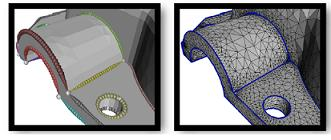On computing handle and tunnel loops T. K. Dey, K. Li, and  J. Sun.  IEEE Proc. Internat. Conf. Cyberworlds (NASAGEM workshop) (2007), 357--366. Journal version is in Computer-Aided Design, in press, doi:10.1016/j.cad.2009.01.001. [Talk slides] HandleTunnel software Many applications seek to identify features like handles' and tunnels' in a shape bordered by a surface embedded in three dimensions. To this end we define handle and tunnel loops on surfaces which can help identifying these features. We show that a closed surface of genus g always has g handle and g tunnel loops induced by the embedding. For a class of shapes that retract to graphs, we characterize these loops by a linking condition with these graphs. These characterizations lead to algorithms for detection and generation of these loops. We provide an impementation with applications to feature detection and topology simplification to show the effectiveness of the method.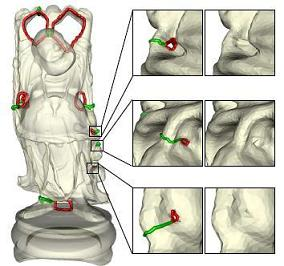Stability of critical points with interval persistence T. K. Dey and R. Wenger.  Discrete & Computational Geometry, vol 38 (2007), 479--512. Tech Report OSU-CISRC-4/06-TR47, April 2006. Scalar functions defined on a topological space T are at the core of many applications such as shape matching, visualization, and physical simulations. Topological persistence is an approach to characterizing these functions. It measures how long topological structures in the level sets {x in T : f(x) <= c} persist as c changes. Recently it was shown that the critical values defining a topological structure with relatively large persistence remain almost unaffected by small perturbations. This result suggests that topological persistence is a good measure for matching and comparing scalar functions. We extend these results to critical points in the domain by redefining persistence and critical points and replacing sub-level sets {x in T : f(x) <= c} with interval sets {x in T : a<=f(x)< b}. With these modifications we establish a stability result for critical points. This result is strengthened for maxima that can be used for matching two scalar functions.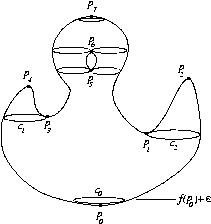Delaunay meshing of isosurfaces T. K. Dey and J. A. Levine.  Proc. Shape Modeling International (SMI 2007), 241--250. DelIso software We present an isosurface meshing algorithm DelIso, based on the Delaunay refinement paradigm. This paradigm has been successfully applied to mesh a variety of domains with guarantees for topology, geometry, mesh gradedness, and triangle shape. A restricted Delaunay triangulation, dual of the intersection between the surface and the three dimensional Voronoi diagram, is often the main ingredient in Delaunay refinement. Computing and storing three dimensional Voronoi/Delaunay diagrams become bottlenecks for Delaunay refinement techniques since isosurface computations generally have large input datasets and output meshes. A highlight of our algorithm is that we find a simple way to recover the restricted Delaunay triangulation of the surface without computing the full 3D structure. We employ techniques for efficient ray tracing of isosurfaces to generate surface sample points, and demonstrate the effectiveness of our implementation on a variety of volume datasets.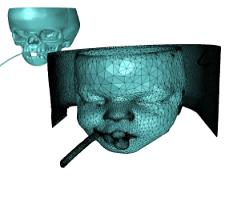Delaunay refinement for piecewise smooth complexes S.-W. Cheng, T. K. Dey, and E. A. Ramos. Proc. 18th Annu. ACM-SIAM Sympos. Discrete Algorithms (SODA 2007), 1096--1105. Journal Version in Discrete & Comput. Geom., 2008, article in press. doi.10.1007/s00454-008-9109-3. Extended Full Version We present a Delaunay refinement algorithm for meshing a piecewise smooth complex in three dimensions with correct topology. The small angles between the tangents of two meeting manifold patches pose difficulty. We protect these regions with weighted points. The weights are chosen to mimic the local feature size and to satisfy a Lipschitz-like property. A Delaunay refinement using the weighted Voronoi diagram is shown to terminate with the recovery of the topology of the input. To this end, we present new concepts and results including a new definition of local feature size and a proof for a generalized topological ball property.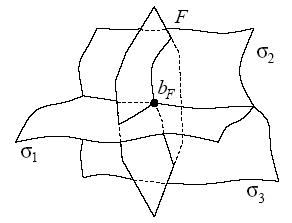Defining and computing curve-skeletons with medial geodesic function T. K. Dey and J. Sun. Proc. Sympos. Geometry Processing (SGP 2006), 143--152. CurveSkel Software Many applications in geometric modeling, computer graphics, visualization, and computer vision benefit from a reduced representation called curve-skeletons of a shape. These are curves possibly with branches which compactly represent the shape geometry and topology. The lack of a proper mathematical definition has been a bottleneck in developing and applying the curve-skeletons. A set of desirable properties of these skeletons has been identified and the existing algorithms try to satisfy these properties mainly through a procedural definition. We define a function called medial geodesic on the medial axis which leads to a mathematical definition and an approximation algorithm for curve-skeletons. Empirical study shows that the algorithm is robust against noise, operates well with a single user parameter, and produces curve-skeletons with the desirable properties. Moreover, the curve-skeletons can be associated with additional attributes that follow naturally from the definition. These attributes capture shape eccentricity, a local measure of how far a shape is away from a tubular one.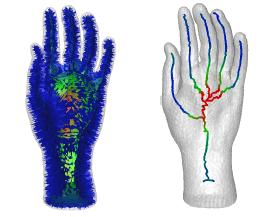Identifying flat and tubular regions of a shape by unstable manifolds S. Goswami, T. K. Dey, and C. Bajaj. Proc. 11th ACM Sympos. Solid Modeling Applications (SPM 2006), 27--37. We present an algorithm to identify the flat and tubular regions of a three dimensional shape from its point sample. We consider the distance function to the input point cloud and the Morse structure induced by it on R^3. Specifically we focus on the index 1 and index 2 saddle points and their unstable manifolds. The unstable manifolds of index 2 saddles are one dimensional whereas those of index 1 saddles are two dimensional. Mapping these unstable manifolds back onto the surface, we get the tubular and flat regions. The computations are carried out on the Voronoi diagram of the input points by approximating the unstable manifolds with Voronoi faces. We demonstrate the performance of our algorithm on several point sampled objects.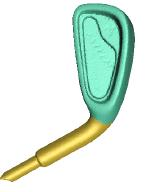Normal and feature approximations from noisy point clouds. T. K. Dey and J. Sun.  Proc. FST&TCS 2006, LNCS 4337,  21--32. NormFet software We consider the problem of approximating normal and feature sizes approximations of a surface from point cloud data that may be noisy. These problems are central to many applications dealing with point cloud data. In the noise-free case, the normals and feature sizes can be approximated by the centers of a set of unique large Delaunay balls called polar balls. In presence of noise, polar balls do not necessarily remain large and hence their centers may not be good for normal and feature approximations. Earlier works suggest that some large Delaunay balls can play the role of polar balls. However, these results were short in explaining how the big Delaunay balls should be chosen for reliable approximations and how the approximation error depends on various factors. We provide new analyses that fill these gaps. In particular, they lead to new algorithms for practical and reliable normal and feature approximations.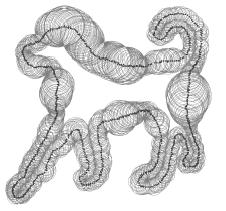Anisotropic surface meshing S.-W. Cheng, T. K. Dey and E. A. Ramos. Proc. ACM-SIAM Sympos. Discrete Algorithms (SODA 2006), 202--211. We study the problem of triangulating a smooth closed implicit surface S endowed with a 2D metric tensor that varies over S. This is commonly known as the anisotropic surface meshing problem. We extend the 2D metric tensor naturally to 3D and employ the 3D anisotropic Voronoi diagram of a set P of samples on S to triangulate S. We prove that a restricted dual, Mesh P,  is a valid triangulation homeomorphic to S under appropriate conditions. We also develop an algorithm for constructing P and Mesh P.  In addition to being homeomorphic to S, each triangle in Mesh P is well-shaped when measured using the 3D metric tensors of its vertices. Users can set upper bounds on the anisotropic edge lengths and the angles between the surface normals at vertices and the normals of incident triangles (measured both isotropically and anisotropically).
 An Adaptive MLS Surface for Reconstruction with Guarantees. T. K. Dey and J. Sun.  Symposium on Geometry Processing (SGP 2005), 43--52. Conference version. Extended version. Technical Report OSU-CISRC-4-05-TR26, April, 2005. AMLS Software Recent work have shown that moving least squares (MLS) surfaces can be used effectively to reconstruct surfaces from possibly noisy point cloud data. Several variants of MLS surfaces have been suggested, some of which have been analyzed theoretically for guarantees. These analyses, so far, have assumed uniform sampling density. We propose a new variant of the MLS surface that, for the first time, incorporates local feature sizes in its formulation, and we analyze it for reconstruction guarantees using a non-uniform sampling density. The proposed variant of the MLS surface has several computational advantages over existing MLS methods. Also see Extremal Surface Based Projections Converge and Reconstruct with Isotopy. T. K. Dey, S. Goswami and J. Sun. Technical Report OSU-CISRC-05-TR25, April, 2005. An early attempt T. K. Dey, S. Goswami and J. Sun. Smoothing noisy point clouds with Delaunay preprocessing and MLS. Tech-report OSU-CISRC-3/04-TR17, 2004.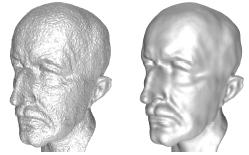Normal Estimation for Point Clouds :  A Comparison Study for a Voronoi Based Method T. K. Dey, G. Li and J. Sun.  Eurographics Sympos. on Point-Based Graphics (2005), 39--46. Many applications that process a point cloud data benefit from a reliable normal estimation step. Given a point cloud presumably sampled from an unknown surface, the problem is to estimate the normals of the surface at the data points. Two approaches, one based on numerical optimizations and another based on Voronoi diagrams are known for the problem. Variations of numerical approaches work well even when point clouds are contaminated with noise. Recently a variation of the Voronoi based method is proposed for noisy point clouds. The centrality of the normal estimation step in point cloud processing begs a thorough study of the two approaches so that one knows which approach is appropriate for what circumstances. This paper presents such results.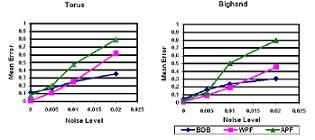Polygonal Surface Remeshing with Delaunay Refinement  T. K. Dey, G. Li and T. Ray. Proc.14th Internat. Meshing Roundtable, (IMR 2005), 343--361. Journal version in Engineering with Computers, vol. 26, page 289--301, 2010. Conference version Extended version SurfRemesh software Polygonal meshes are used to model smooth surfaces in many applications. Often these meshes need to be remeshed for improving the quality, density or gradedness. We apply the Delaunay refinement paradigm to design a provable algorithm for isotropic remeshing of a polygonal mesh that approximates a smooth surface. The proofs provide new insights and our experimental results corroborate the theory.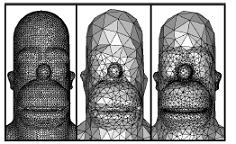Weighted Delaunay Refinement for Polyhedra with Small Angles S.-W. Cheng, T. K. Dey and T. Ray. Proc.14th Internat. Meshing Roundtable  (IMR 2005), 325--342. Recently, a provable Delaunay meshing algorithm called QMesh has been proposed for polyhedra that may have acute input angles. The algorithm guarantees bounded circumradius to shortest edge length ratio for all tetrahedra except the ones near small input angles. This guarantee eliminates or limits the occurrences of all types of poorly shaped tetrahedra except slivers. A separate technique called weight pumping is known for sliver elimination. But, allowable input for the technique so far have been periodic point sets and piecewise linear complex with non-acute input angles. In this paper, we incorporate the weight pumping method into QMesh thereby ensuring that all tetrahedra except the ones near small input angles have bounded aspect ratio. Theoretically, the algorithm has an abysmally small angle guarantee inherited from the weight pumping method. Nevertheless, our experiments show that it produces better angles in practice.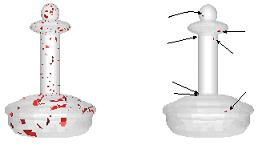Critical points of distance to an epsilon-sampling of a surface and flow-complex- based surface reconstruction. T. K. Dey, J. Giesen, E. A. Ramos and B. Sadri. Proc. 21st Annu. Sympos. Comput.  Geom., (SOCG 2005), 218--227. ( Invited Journal version in Internat. J. Comput. Geom. Applications, Vol 18, 2008, 29--61.) Extended version. The distance function to surfaces in three dimensions plays a key role in many geometric modeling applications such as medial axis approximations, surface reconstructions, offset computations, feature extractions and others. In most of these cases, the distance function to the surface is approximated by a discrete distance function where the distances are measured from a discrete sample of the surface. The critical points of the distance function determines the topology of the geometric structures in question. Howvere, no theoretical result exists linking the sampling and the critical point structures of the distance functions. We provide this link by showing that the critical points of the discrete distance function either lie very close to the surface or near the medial axis both quantified with the sampling density. One implication of this result is that known Morse theory based surface reconstruction algorithms do indeed approximate the surface geometrically.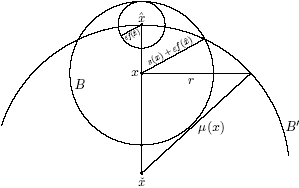Manifold Reconstruction from Point Samples Siu-Wing Cheng, T. K. Dey and E. A. Ramos. Proc. ACM-SIAM Sympos. Discrete Algorithms, (SODA 2005), 1018--1027. Extended AbstractSee also [Talk slides] We present an algorithm to "reconstruct" a smooth k-dimensional manifold M embedded in an Euclidean space R^d from a "sufficiently dense" point sample from the manifold. The algorithm outputs a simplicial manifold that is homeomorphic and geometrically close to M. The running time is O(nlogn) where n is the number of points in the sample (the multiplicative constant depends exponentially on the dimension though).Delaunay Triangulations Approximate Anchor Hulls T. K. Dey, Joachim Giesen and Samrat Goswami. Comput. Geom. Theory Applications, vol. 36 (2006), 131--143. (prelim. version in (SODA 2005), 1028-1037). Recent results establish that a subset of the Voronoi diagram of a point set that is sampled from a smooth boundary of a shape approximates the medial axis. The corresponding question for the dual Delaunay triangulation is not addressed in the literature. We show that, for two dimensional shapes, the Delaunay triangulation approximates a specific structure which we call anchor hulls. As an application we demonstrate that our approximating result is useful for the problem of shape matching.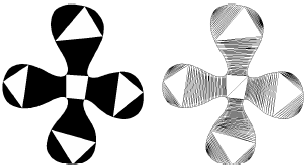Quality meshing for polyhedra with small angles S.-W. Cheng, T. K. Dey, E. Ramos and T. Ray. International J. Comput. Geom. & Applications, Vol. 15, No. 4 (2005),  421--461. Prelim. version in (SOCG 2004). Extended version QualMesh Software We present an algorithm to compute a Delaunay mesh conforming to a polyhedron possibly with small input angles. The radius-edge ratio of most output tetrahedra are bounded by a constant, except possibly those that are provably close to small angles. Further, the mesh is graded, that is, edge lengths are at least a constant fraction of the local feature sizes at the edge endpoints. Unlike a previous algorithm, this algorithm is simple to implement as it avoids computing local feature sizes and protective zones explicitly. Our experimental results confirm our claims and show that few skinny tetrahedra remain.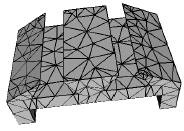Sampling and meshing a surface with guaranteed topology and geometry S.-W. Cheng, T. K. Dey, E. Ramos and T. Ray.Proc. 20th Sympos. Comput. Geom. (SOCG 2004), 280--289 . Extended version,  in SIAM Journal Computing, Vol. 37 (2007), 1199--1227. This paper presents an algorithm for sampling and triangulating a smooth surface in R3 where the triangulation is homeomorphic to the surface. The only assumption we make is that the input surface representation is amenable to certain types of computations, namely computations of the intersection points of a line with the surface, computations of the critical points of some height functions defined on the surface and its restriction to a plane, and computations of some silhouette points. The algorithm ensures bounded aspect ratio, size optimality, and smoothness of the output triangulation. Unlike previous algorithms, this algorithm does not need to compute the local feature size for generating the sample points which was a major bottleneck. Experiments show the usefulness of the algorithm in remeshing and meshing CAD surfaces that are piecewise smooth.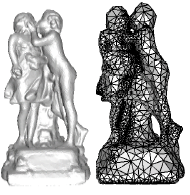Provable surface reconstruction from noisy samples T. K. Dey and S. Goswami. Computationa Geometry Theory & Applications, vol. 35, 2006, 124--141. Prelim. version in (SOCG 2004). RobustCocone software We present an algorithm for surface reconstruction in presence of noise. We show that, under a reasonable noise model, the algorithm has theoretical guarantees. Actual performance of the algorithm is illustrated by our experimental results.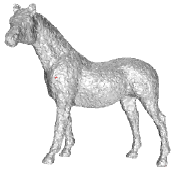Approximating the Medial Axis from the Voronoi Diagram with a Convergence Guarantee T. K. Dey and W. Zhao. Algorithmica, vol. 38, 2003, 179--200. Medial Software The medial axis of a surface in 3D is the closure of all points that have two or more closest points on the surface. It is an essential geometric structure in a number of applications involving 3D geometric shapes. Since exact computation of the medial axis is difficult in general, efforts continue to improve their approximations. Voronoi diagrams turn out to be useful for this approximation. Although it is known that Voronoi vertices for a sample of points from a curve in 2D approximate its medial axis, similar result does not hold in 3D. Recently, it has been discovered that only a subset of Voronoi vertices converge to the medial axis as sample density approaches infinity. However, most applications need a non-discrete approximation as opposed to a discrete one. To date no known algorithm can compute this approximation straight from the Voronoi diagram with a guarantee of convergence.We present such an algorithm and its convergence analysis in this paper. One salient feature of the algorithm is that it is scale and density independent. Experimental results corroborate our theoretical claims.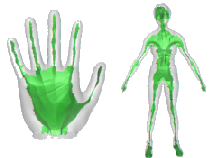Hierarchy of surface models and irreducible triangulation S.-W. Cheng, T. K. Dey and S.-H. Poon.Computational Geometry : Theory & Applications, Vol. 27, No. 2 (2004), 135--150 Given a triangulated closed surface, the problem of constructing a hierarchy of surface models of decreasing level of detail has attracted much attention in computer graphics. A hierarchy provides a view-dependent refinement and facilitates the computation of parameterization. For a triangulated closed surface of n vertices and genus g, we prove that there is a constant c>0 such that if n > c g, a greedy strategy can identify \Theta(n) topology-preserving edge contractions that do not interfere with each other. Further, each of them affects only a constant number of triangles. Repeatedly identifying and contracting such edges produces a topology-preserving hierarchy of O(n+g^2) size and O(logn +g) depth. Although several implementations exist for constructing hierarchies, our work is the first to show that a greedy algorithm can efficiently compute a hierarchy of provably small size and low depth. When no contractible edge exists, the triangulation is irreducible. Nakamoto and Ota showed that any irreducible triangulation of an orientable 2-manifold has at most max{342g-72, 4} vertices. Uisng our proof techniques we obtain a new bound of mx{240g,4}.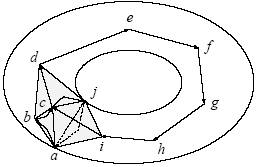Shape segmentation and matching with flow discretization T. K. Dey, J. Giesen and S. Goswami. Proc. Workshop Algorithms Data Strucutres (WADS 03), LNCS 2748, F. Dehne, J.-R. Sack, M. Smid Eds., 25--36 Extended version Segmatch Software Geometric shapes are identified with their features. For computational purposes a concrete mathematical definition of features is required. In this paper we use a topological approach, namely dynamical systems, to define features of shapes. To exploit this definition algorithmically we assume that a point sample of the shape is given as input from which features of the shape have to be approximated. We translate our definition of features to the discrete domain while mimicking the set-up developed for the continuous shapes. The outcome of this approach is a clean mathematical definition of features that are efficiently computable with combinatorial algorithms. Experimental results show that our algorithms segment shapes in two and three dimensions into so-called features quite effectively. Further, we develop a shape matching algorithm that takes advantage of our robust feature segmentation step. Performance of this algorithm is exhibited with experimental results. Also see the following paper: Shape segmentation and matching from noisy point clouds T. K. Dey, J. Giesen and S. Goswami. Proc. Eurographics Sympos. Point-Based Graphics (2004), Marc Alexa and S. Rusinkiewicz (eds) (2004), 193--199.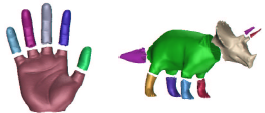Quality meshing with weighted Delaunay refinement S.-W. Cheng and T. K. Dey.SIAM J. Computing, Vol. 33 (2003), 69--93 Preliminary version in (SODA 2002). Delaunay meshes with bounded circumradius to shorten edge length ratio have been propsed in the past for quality meshing. The only poor quality tetrahedra called slivers that can occur in such a mesh can be eliminated by the sliver exudation method. This method has been shown to work for periodic point sets, but not with boundaries. Recently a randomized point-placement strategy has been proposed to remove slivers while conforming to a given boundary. In this paper we present a deterministic algorithm for generating a weighted Delaunay mesh which respects the input boundary and has no poor quality tetrahedron including slivers. As in previous work, we assume that no input angle is acute. This success is achieved by combining the weight pumping method for sliver exudation and the Delaunay refinement method for boundary conformation.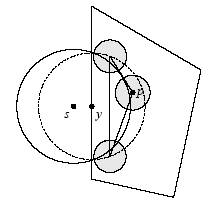Shape dimension and approximation from samples T. K. Dey, J. Giesen, S. Goswami and W. Zhao. Discrete & Comput. Geom., vol 29, 419--434 (2003)  Prelim. version in (SODA 2002). There are many scientific and engineering applications where an automatic detection of shape dimension from sample data is necessary. Topological dimensions of shapes constitute an important global feature of them. We present a Voronoi based dimension detection algorithm that assigns a dimension to a sample point which is the topological dimension of the manifold it belongs to. Based on this dimension detection, we also present an algorithm to approximate shapes of arbitrary dimension from their samples. Our empirical results with data sets in three dimensions support our theory.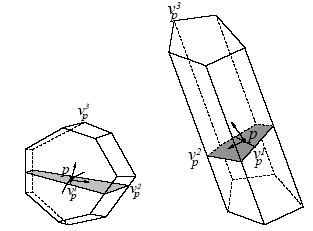A simple algorithm for homeomorphic surface reconstruction N. Amenta, S. Choi, T. K. Dey and N. Leekha. Intl. Journal on Computational Geometry & Applications, vol. 12, (2002), 125--141. Prelim. version in (SOCG 2000). The problem of computing a piecewise linear approximation to a surface from a set of sample points is improtant in solid modeling, computer graphics and computer vision. A recent algorithm using the Voronoi diagram of the sample points gave a guarantee on the distance of the output surface from the original sampled surface assuming that the sample was sufficiently dense. We give a similar algorithm, simplifying the computation and the proof of the geometric guarantee. In addition, we guarantee that our output surface is homeomorphic to the original surface; to our knowledge this is the first such topological guarantee for this problem. Also see the following paper: Tight Cocone : A water-tight surface reconstructor T. K. Dey and S. Goswami. J. Computing Inform. Sci. Engin., vol. 30, (2003), 302--307. Cocone Software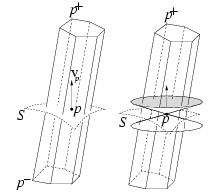Dynamic skin triangulation H-L. Cheng, T. K. Dey, H. Edelsbrunner and J. Sullivan. Discrete Comput. Geom., vol. 25, (2001), 525--568 Prelim. version in (SODA 2001). This paper describes an algorithm for maintaining an approximating triangulation of a deforming surface in R 3 . The surface is the envelope of an infinite family of spheres defined and controlled by a finite collection of weighted points. The triangulation adapts dynamically to changing shape, curvature, and topology of the surface.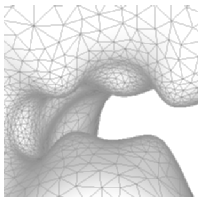Sliver exudation S.-W. Cheng, T. K. Dey, H. Edelsbrunner, M. A. Facello and S.-H. Teng.J. ACM, Vol. 47, (2000), 883--904 Prelim. version in (SOCG 1999). A sliver is a tetrahedron whose four vertices lie close to a plane and whose orthogonal projection to that plane is a convex quadrilateral with no short edge. Slivers are notoriously common in 3-dimensional Delaunay triangulations even for well-spaced point sets. We show that if the Delaunay triangulation has the ratio property then there is an assignment of weights so the weighted Delaunay triangulation contains no slivers. We also give an algorithm to compute such a weight assignment.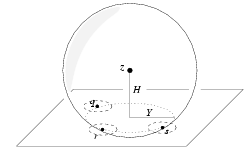A simple provable algorithm for curve reconstruction T. K. Dey and P. Kumar.Proc. 10th. ACM-SIAM Symposium on Discrete Algorithms (SODA '99) , 1999, 893--894 We present an algorithm that provably reconstructs a curve in the framework introduced by Amenta, Bern and Eppstein. The highlights of the algorithm are: (i) it is simple, (ii) it requires a sampling density better than previously known, (iii) it can be adapted for curve reconstruction in higher dimensions straightforwardly.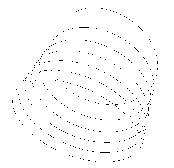Topology preserving edge contraction T. K. Dey, H. Edelsbrunner, S. Guha and D. Nekhayev.Publications de l' Institut Mathematique (Beograd), Vol. 60 (80), 23--45, 1999 We study edge contractions in simplicial complexes and local conditions under which they preserve the topological type. The conditions are based on a generalized notion of boundary, which lends itself to defining a nested hierarchy of triangulable spaces measuring the distance to being a manifold.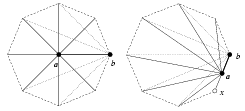Transforming curves on surfaces T. K. Dey and S. Guha.Journal of Computer and System Sciences, vol. 58, 1999, 297--325. Preliminary version in IEEE (FOCS 1995), 266-274 We describe an optimal algorithm to decide if one closed curve on a triangulated 2-manifold can be continuously transformed to another, i.e., if they are homotopic. Suppose C_1 and C_2 are two closed curves on a surface M of genus g. Further, suppose T is a triangulation of M of size n such that C_1 and C_2 are represented as edge-vertex sequences of lengths k_1 and k_2 in T, respectively. Then, our algorithm decides if C_1 and C_2 are homotopic in O(n+k_1+k_2) time and space, provided g \not = 2 if M is orientable, and g \not 3, 4 if M is non-orientable. This as well implies an optimal algorithm to decide if a closed curve on a surface can be continuously contracted to a point. Except for the three low genus cases, our algorithm completes an investigation into the computational complexity of two classical problems for surfaces posed by the mathematician Max Dehn at the beginning of this century. The novelty of our approach is in the application of methods from modern combinatorial group theory. Reprint available upon request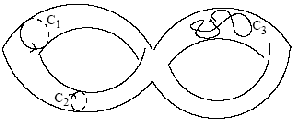Improved bounds for planar k-sets and related problems T. K. Dey.Invited paper in a special issue of Discrete & Computational Geometry, Vol. 19, No. 3, (1998), 373-382 Prelim. version in IEEE (FOCS 1997). We prove an O(nk1/3) upper bound for planar k-sets. This is the first considerable improvement on this bound after its early solutions approximately twenty seven years ago. Our proof technique also applies to improve the current bounds on the combinatorial complexities of k-levels in arrangements of line segments, k convex polygons in the union of n lines, parametric minimum spanning trees and parametric matroids in general.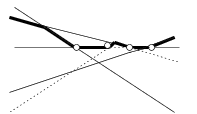Extremal problems for geometric hypergraphs T. K. Dey and J. Pach.Discrete & Computational Geometry, Vol. 19, No. 4, (1998), 473--484. Preliminary version in ISAAC 96, LNCS 1178, 105-114 A geometric hypergraph H is a collection of i-dimensional simplices, called hyperedges or, simply, edges, induced by some (i + 1)-tuples of a vertex set V in general position in d-space. The topological structure of geometric graphs, i.e., the case d = 2; i = 1, has been studied extensively, and it proved to be instrumental for the solution of a wide range of problems in combinatorial and computational geometry. They include the k-set problem, proximity questions, bounding the number of incidences between points and lines, designing various efficient graph drawing algorithms, etc. In this paper, we make an attempt to generalize some of these tools to higher dimensions. We will mainly consider extremal problems of the following type. What is the largest number of edges (i-simplices) that a geometric hypergraph of n vertices can have without containing certain forbidden configurations? In particular, we discuss the special cases when the forbidden configura� tions are k intersecting edges, k pairwise intersecting edges, k crossing edges, k pairwise crossing edges, k edges that can be stabbed by an i-flat, etc. Some of our estimates will be tight.
 Computational Topology T. K. Dey, H. Edelsbrunner and S. Guha. Advances in Discrere and Computational Geometry, eds. B. Chazelle, J. E. Goodman and R. Pollack. Contemporary Mathematics, AMS, Providence, 1998 . The authors of this article believe there is or should be a research area appropriately referred to as computational topology. Its agenda includes the identification and formalization of topological questions in computer applications and the study of algorithms for topological problems. It is hoped this article can contribute to the creation of a computational branch of topology with a unifying influence on computing and computer applications.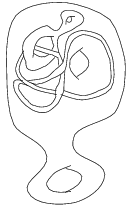Visibility with multiple reflections B. Aronov, A. Davis, T. K. Dey, S. P. Pal and D. C. Prasad.Discrete & Computational Geometry, Vol. 20, No. 61, (1998), 61--78. Preliminary version in 5th SWAT, 1996, LNCS 1097, 284-295 We show that the region lit by a point light source inside a simple n-gon after at most k reflections off the boundary has combinatorial complexity O(n2k ), for any k – 1. A lower bound of Ω((n/k θ(1))2k ) is also established which matches the upper bound for any fixed k. A simple near-optimal algorithm for computing the illuminated region is presented, which runs in O(n2k log n) time and O(n2k ) space for k > 1, and in O(n2 log2 n) time and O(n2 ) space for k = 1.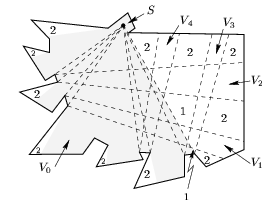Counting triangle crossings and halving planes T. K. Dey and H. Edelsbrunner.Invited paper in a special issue, Discrete & Computational Geometry, Vol. 12 (1994), 281--289 Prelim. version in SoCG 1995. Every collection of t > 2n^2 triangles with a total of n vertices in R^3 has \Omega(t^4/n^6) crossing pairs. This implies that one of their edges meets \Omega(t^3/n^6) of the triangles. From this it follows that n points in R^3 have only O(n^{8/3}) halving planes. Reprint available upon request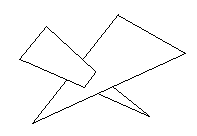On counting triangulations in d dimensions T. K. Dey. Computational Geometry: Theory and Applications, Vol. 3 (1993), 315--325. Given a set of n labeled points on S^d, how many combinatorially different geometric triangulations for this point set are there? We show that the logarithm of this number is at most some positive constant times n^{\floor d/2}+1. Evidence is provided that for even dimensions d the bound can be improved to some constant times n^{d/2}.

Tamal K. Dey's Webpage